Скачать презентацию Chapter 15 Required Returns and the Cost of

8a3b25a61b3bec115ed6cf5d2758bf29.ppt

• Количество слайдов: 68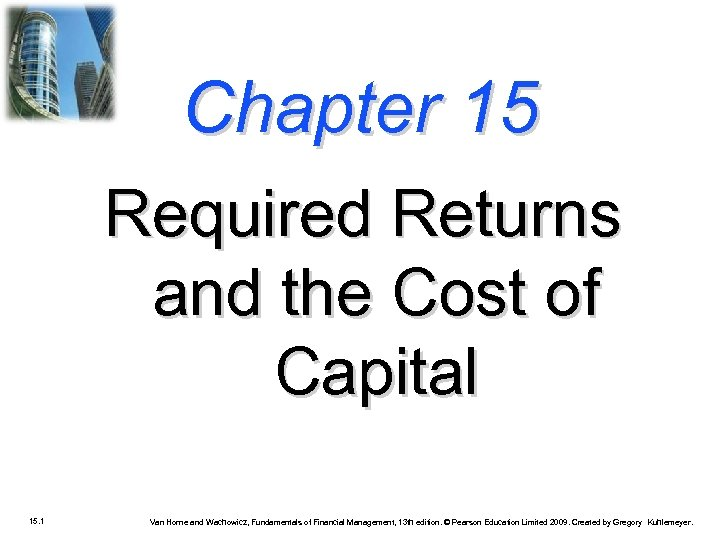Chapter 15 Required Returns and the Cost of Capital 15. 1 Van Horne and Wachowicz, Fundamentals of Financial Management, 13 th edition. © Pearson Education Limited 2009. Created by Gregory Kuhlemeyer.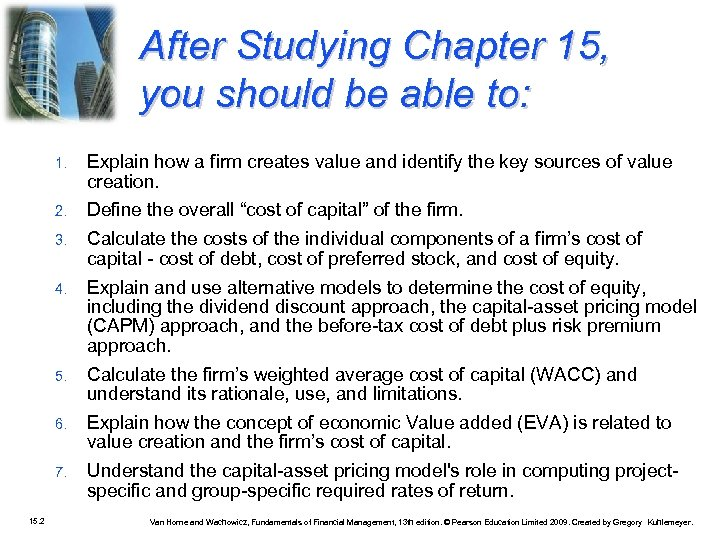After Studying Chapter 15, you should be able to: 1. 2. Define the overall “cost of capital” of the firm. 3. Calculate the costs of the individual components of a firm’s cost of capital - cost of debt, cost of preferred stock, and cost of equity. 4. Explain and use alternative models to determine the cost of equity, including the dividend discount approach, the capital-asset pricing model (CAPM) approach, and the before-tax cost of debt plus risk premium approach. 5. Calculate the firm’s weighted average cost of capital (WACC) and understand its rationale, use, and limitations. 6. Explain how the concept of economic Value added (EVA) is related to value creation and the firm’s cost of capital. 7. 15. 2 Explain how a firm creates value and identify the key sources of value creation. Understand the capital-asset pricing model's role in computing projectspecific and group-specific required rates of return. Van Horne and Wachowicz, Fundamentals of Financial Management, 13 th edition. © Pearson Education Limited 2009. Created by Gregory Kuhlemeyer.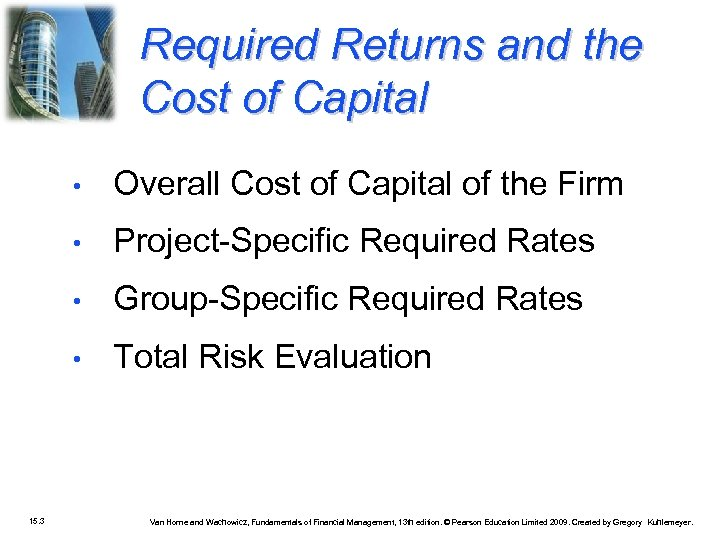Required Returns and the Cost of Capital • • Project-Specific Required Rates • Group-Specific Required Rates • 15. 3 Overall Cost of Capital of the Firm Total Risk Evaluation Van Horne and Wachowicz, Fundamentals of Financial Management, 13 th edition. © Pearson Education Limited 2009. Created by Gregory Kuhlemeyer.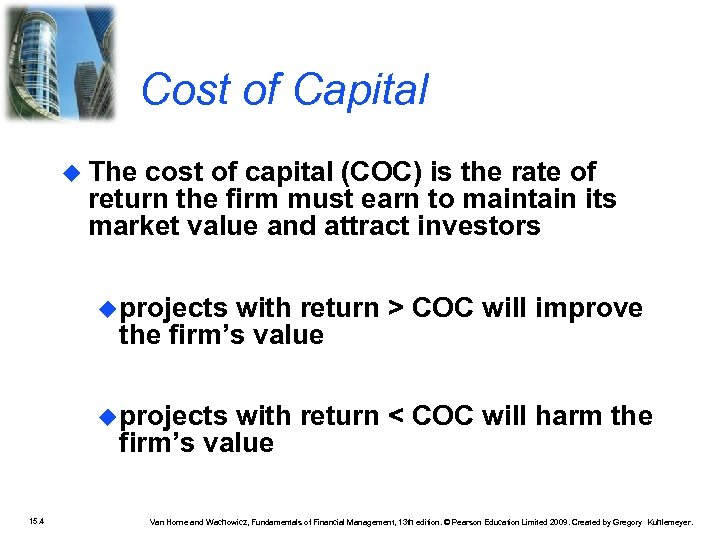Cost of Capital The cost of capital (COC) is the rate of return the firm must earn to maintain its market value and attract investors projects with return > COC will improve the firm’s value projects with return < COC will harm the firm’s value 15. 4 Van Horne and Wachowicz, Fundamentals of Financial Management, 13 th edition. © Pearson Education Limited 2009. Created by Gregory Kuhlemeyer.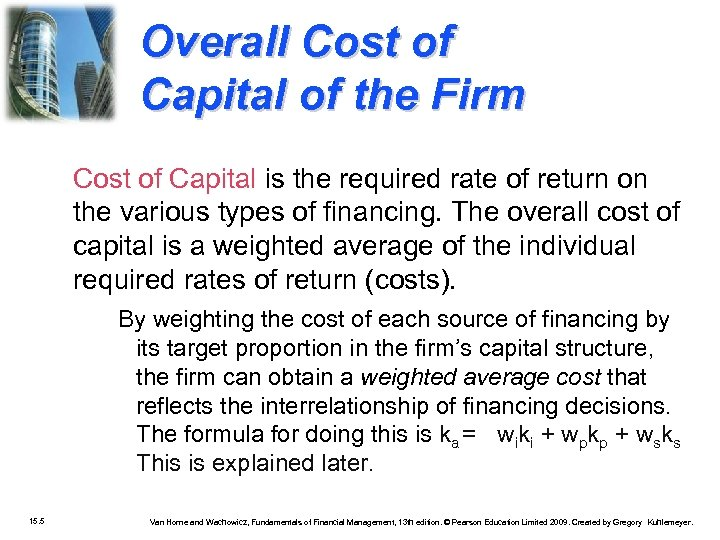Overall Cost of Capital of the Firm Cost of Capital is the required rate of return on the various types of financing. The overall cost of capital is a weighted average of the individual required rates of return (costs). By weighting the cost of each source of financing by its target proportion in the firm’s capital structure, the firm can obtain a weighted average cost that reflects the interrelationship of financing decisions. The formula for doing this is ka = wiki + wpkp + wsks This is explained later. 15. 5 Van Horne and Wachowicz, Fundamentals of Financial Management, 13 th edition. © Pearson Education Limited 2009. Created by Gregory Kuhlemeyer.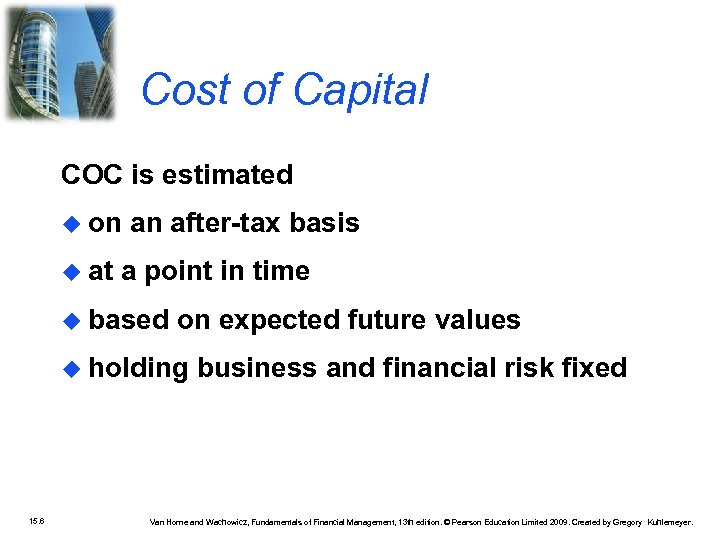Cost of Capital COC is estimated on at an after-tax basis a point in time based on expected future values holding 15. 6 business and financial risk fixed Van Horne and Wachowicz, Fundamentals of Financial Management, 13 th edition. © Pearson Education Limited 2009. Created by Gregory Kuhlemeyer.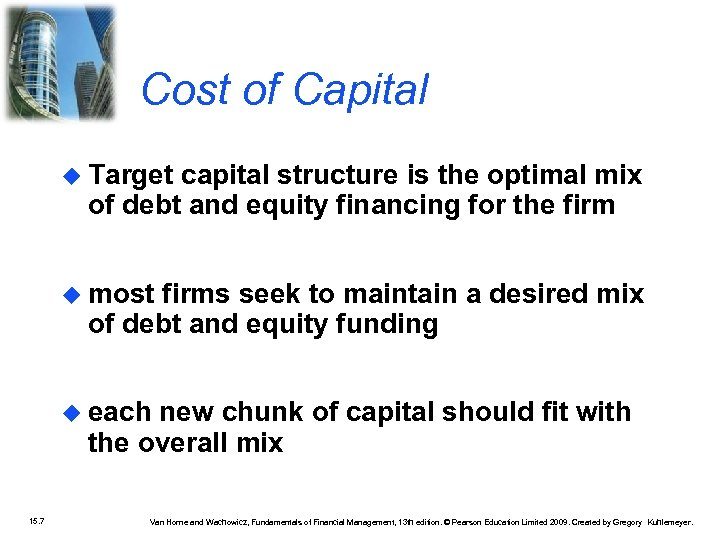Cost of Capital Target capital structure is the optimal mix of debt and equity financing for the firm most firms seek to maintain a desired mix of debt and equity funding each new chunk of capital should fit with the overall mix 15. 7 Van Horne and Wachowicz, Fundamentals of Financial Management, 13 th edition. © Pearson Education Limited 2009. Created by Gregory Kuhlemeyer.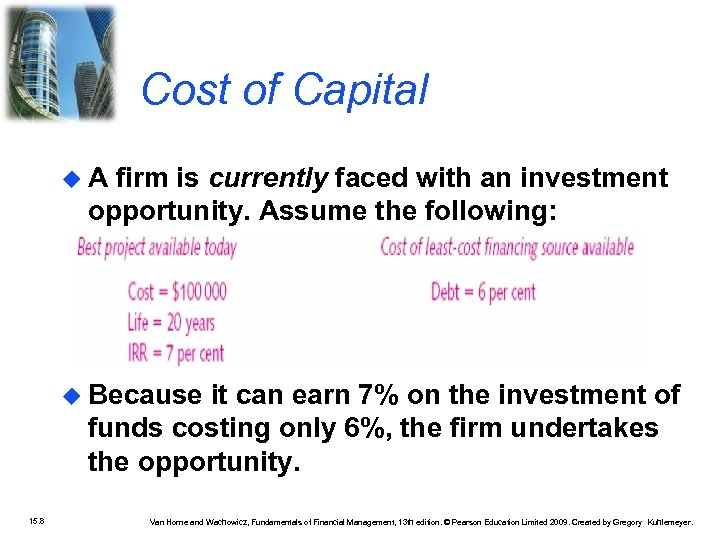Cost of Capital A firm is currently faced with an investment opportunity. Assume the following: Because it can earn 7% on the investment of funds costing only 6%, the firm undertakes the opportunity. 15. 8 Van Horne and Wachowicz, Fundamentals of Financial Management, 13 th edition. © Pearson Education Limited 2009. Created by Gregory Kuhlemeyer.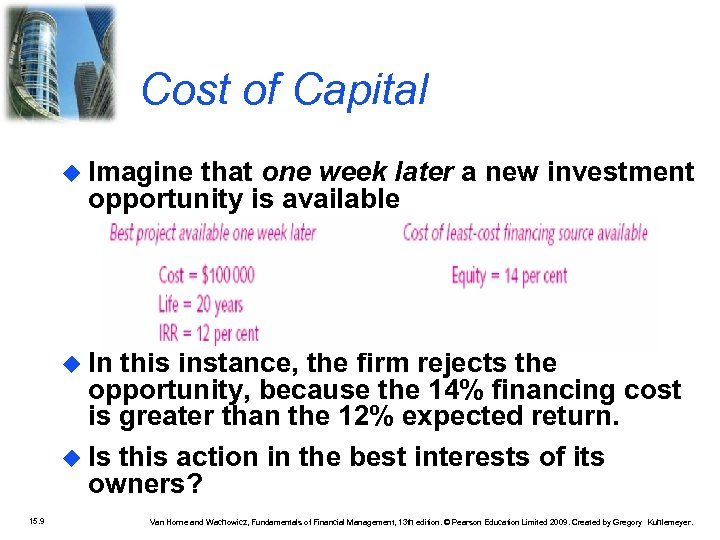Cost of Capital Imagine that one week later a new investment opportunity is available In this instance, the firm rejects the opportunity, because the 14% financing cost is greater than the 12% expected return. Is this action in the best interests of its owners? 15. 9 Van Horne and Wachowicz, Fundamentals of Financial Management, 13 th edition. © Pearson Education Limited 2009. Created by Gregory Kuhlemeyer.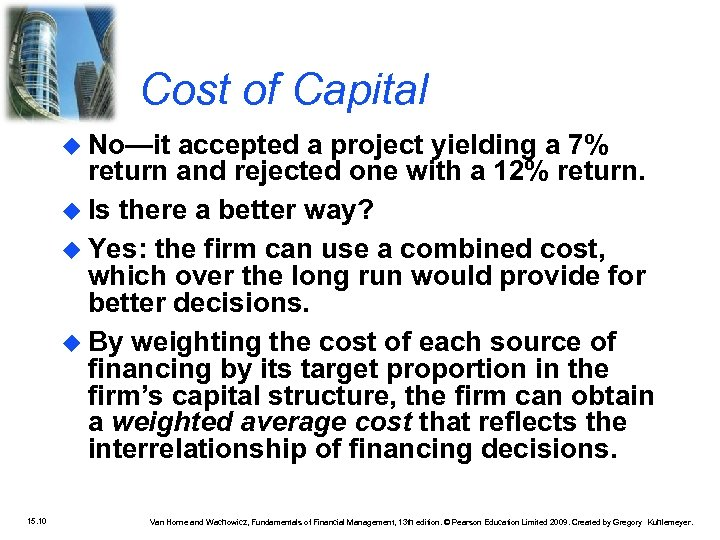Cost of Capital No—it accepted a project yielding a 7% return and rejected one with a 12% return. Is there a better way? Yes: the firm can use a combined cost, which over the long run would provide for better decisions. By weighting the cost of each source of financing by its target proportion in the firm’s capital structure, the firm can obtain a weighted average cost that reflects the interrelationship of financing decisions. 15. 10 Van Horne and Wachowicz, Fundamentals of Financial Management, 13 th edition. © Pearson Education Limited 2009. Created by Gregory Kuhlemeyer.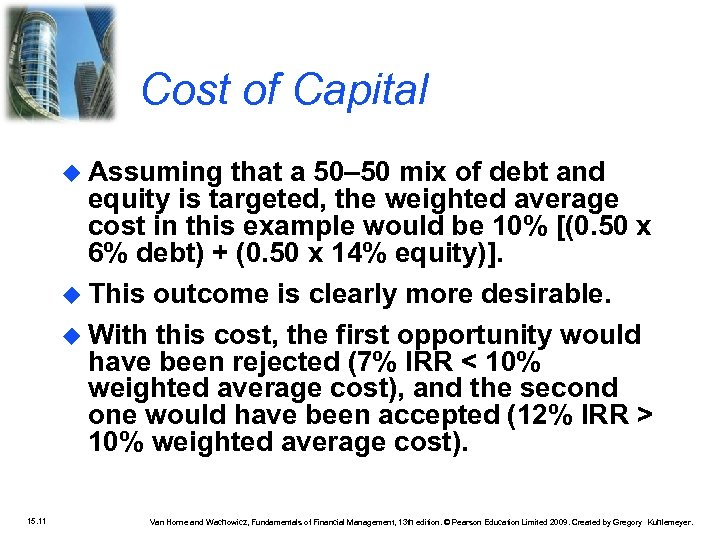Cost of Capital Assuming that a 50– 50 mix of debt and equity is targeted, the weighted average cost in this example would be 10% [(0. 50 x 6% debt) + (0. 50 x 14% equity)]. This outcome is clearly more desirable. With this cost, the first opportunity would have been rejected (7% IRR < 10% weighted average cost), and the second one would have been accepted (12% IRR > 10% weighted average cost). 15. 11 Van Horne and Wachowicz, Fundamentals of Financial Management, 13 th edition. © Pearson Education Limited 2009. Created by Gregory Kuhlemeyer.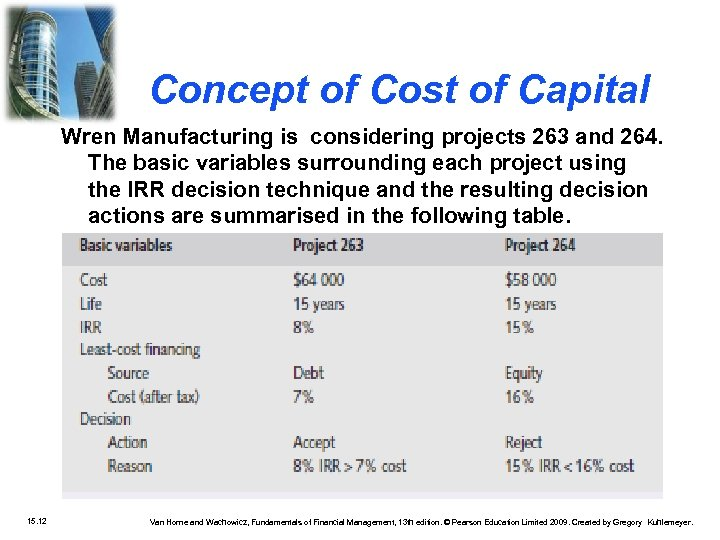Concept of Cost of Capital Wren Manufacturing is considering projects 263 and 264. The basic variables surrounding each project using the IRR decision technique and the resulting decision actions are summarised in the following table. 15. 12 Van Horne and Wachowicz, Fundamentals of Financial Management, 13 th edition. © Pearson Education Limited 2009. Created by Gregory Kuhlemeyer.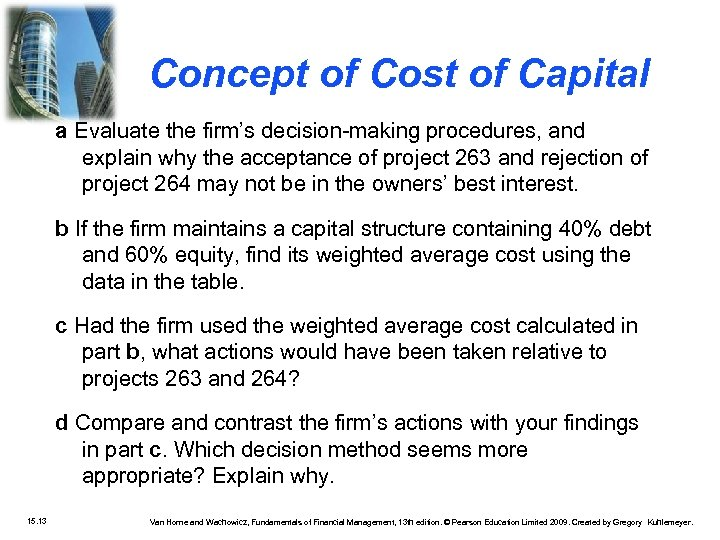Concept of Cost of Capital a Evaluate the firm’s decision-making procedures, and explain why the acceptance of project 263 and rejection of project 264 may not be in the owners’ best interest. b If the firm maintains a capital structure containing 40% debt and 60% equity, find its weighted average cost using the data in the table. c Had the firm used the weighted average cost calculated in part b, what actions would have been taken relative to projects 263 and 264? d Compare and contrast the firm’s actions with your findings in part c. Which decision method seems more appropriate? Explain why. 15. 13 Van Horne and Wachowicz, Fundamentals of Financial Management, 13 th edition. © Pearson Education Limited 2009. Created by Gregory Kuhlemeyer.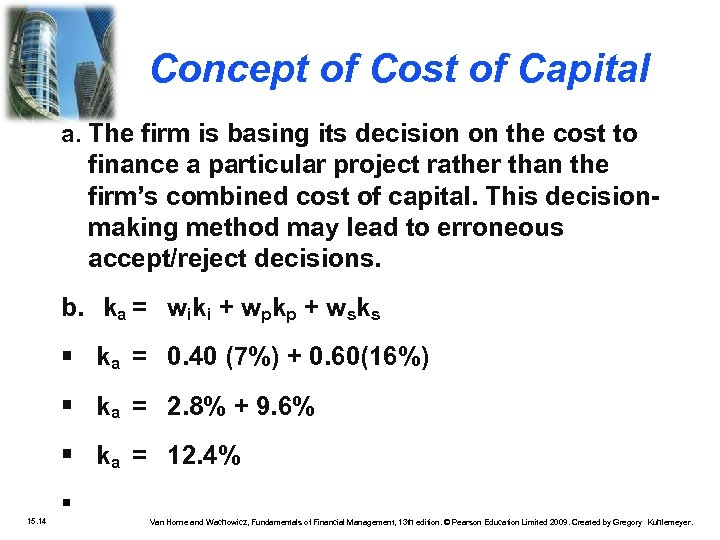Concept of Cost of Capital a. The firm is basing its decision on the cost to finance a particular project rather than the firm’s combined cost of capital. This decisionmaking method may lead to erroneous accept/reject decisions. b. ka = wiki + wpkp + wsks ka = 0. 40 (7%) + 0. 60(16%) ka = 2. 8% + 9. 6% ka = 12. 4% 15. 14 Van Horne and Wachowicz, Fundamentals of Financial Management, 13 th edition. © Pearson Education Limited 2009. Created by Gregory Kuhlemeyer.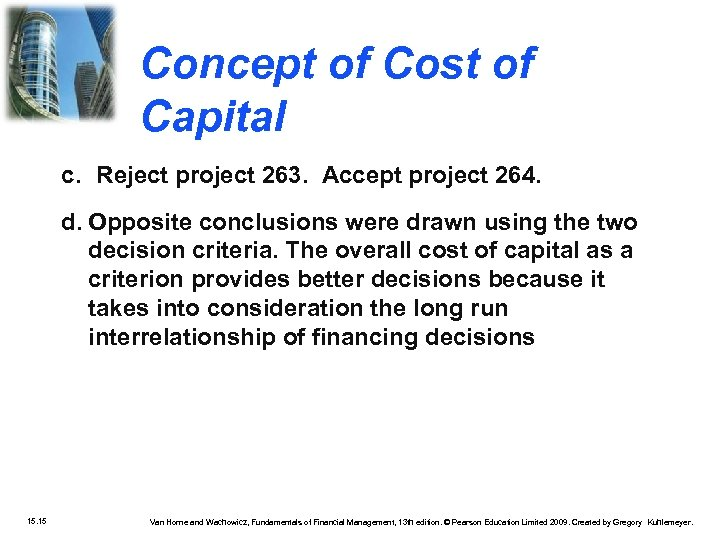Concept of Cost of Capital c. Reject project 263. Accept project 264. d. Opposite conclusions were drawn using the two decision criteria. The overall cost of capital as a criterion provides better decisions because it takes into consideration the long run interrelationship of financing decisions 15. 15 Van Horne and Wachowicz, Fundamentals of Financial Management, 13 th edition. © Pearson Education Limited 2009. Created by Gregory Kuhlemeyer.Sources of finance • • The four sources of long-term funds for the business firm: debt, preference share capital, ordinary share equity capital and • retained earnings. 15. 16 Van Horne and Wachowicz, Fundamentals of Financial Management, 13 th edition. © Pearson Education Limited 2009. Created by Gregory Kuhlemeyer.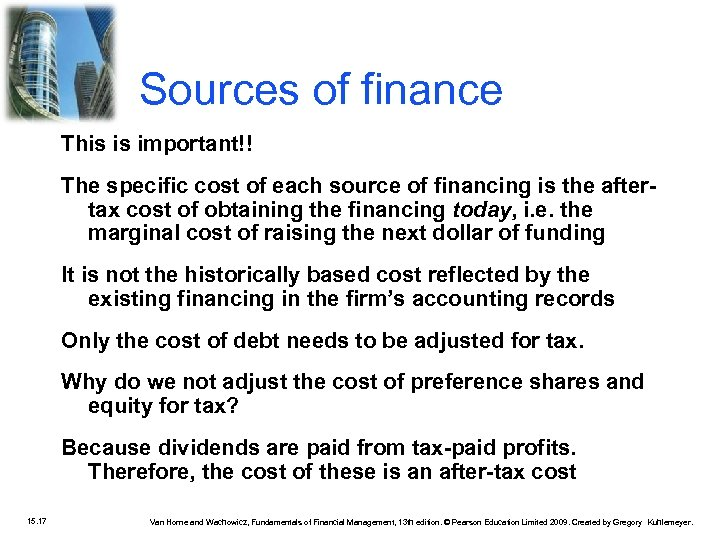Sources of finance This is important!! The specific cost of each source of financing is the aftertax cost of obtaining the financing today, i. e. the marginal cost of raising the next dollar of funding It is not the historically based cost reflected by the existing financing in the firm’s accounting records Only the cost of debt needs to be adjusted for tax. Why do we not adjust the cost of preference shares and equity for tax? Because dividends are paid from tax-paid profits. Therefore, the cost of these is an after-tax cost 15. 17 Van Horne and Wachowicz, Fundamentals of Financial Management, 13 th edition. © Pearson Education Limited 2009. Created by Gregory Kuhlemeyer.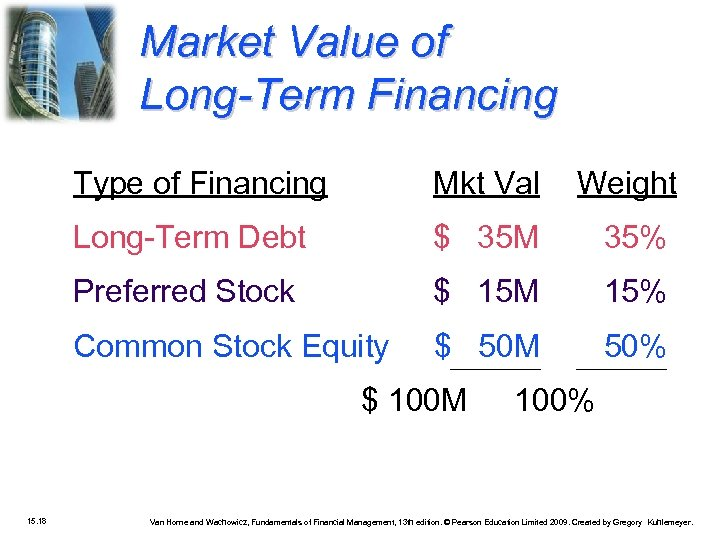Market Value of Long-Term Financing Type of Financing Mkt Val Weight Long-Term Debt \$ 35 M 35% Preferred Stock \$ 15 M 15% Common Stock Equity \$ 50 M 50% \$ 100 M 15. 18 100% Van Horne and Wachowicz, Fundamentals of Financial Management, 13 th edition. © Pearson Education Limited 2009. Created by Gregory Kuhlemeyer.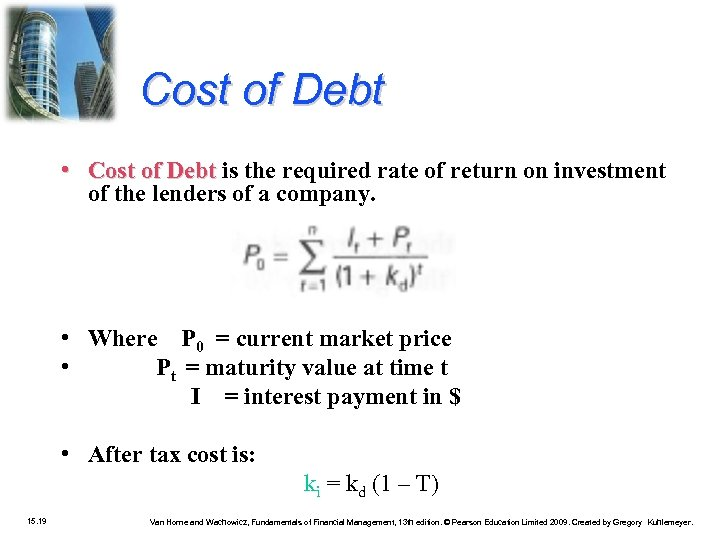Cost of Debt • Cost of Debt is the required rate of return on investment of the lenders of a company. • Where P 0 = current market price • Pt = maturity value at time t I = interest payment in \$ • After tax cost is: ki = kd (1 – T) 15. 19 Van Horne and Wachowicz, Fundamentals of Financial Management, 13 th edition. © Pearson Education Limited 2009. Created by Gregory Kuhlemeyer.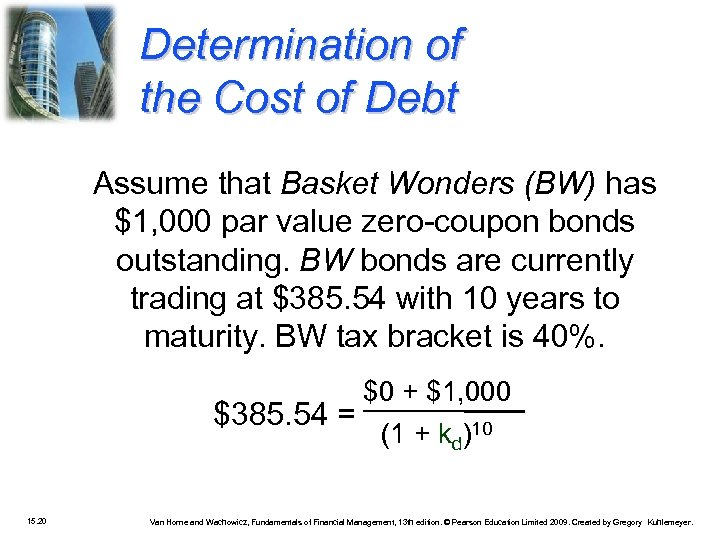Determination of the Cost of Debt Assume that Basket Wonders (BW) has \$1, 000 par value zero-coupon bonds outstanding. BW bonds are currently trading at \$385. 54 with 10 years to maturity. BW tax bracket is 40%. \$0 + \$1, 000 \$385. 54 = (1 + kd)10 15. 20 Van Horne and Wachowicz, Fundamentals of Financial Management, 13 th edition. © Pearson Education Limited 2009. Created by Gregory Kuhlemeyer.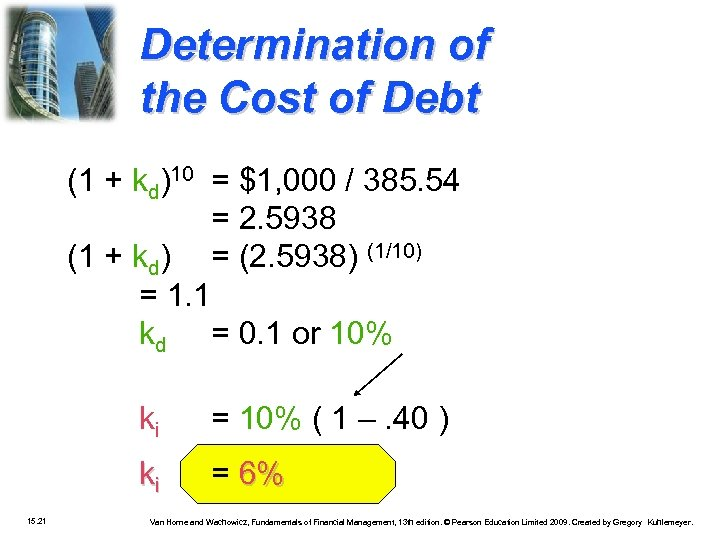Determination of the Cost of Debt (1 + kd)10 = \$1, 000 / 385. 54 = 2. 5938 (1 + kd) = (2. 5938) (1/10) = 1. 1 kd = 0. 1 or 10% ki ki 15. 21 = 10% ( 1 –. 40 ) = 6% Van Horne and Wachowicz, Fundamentals of Financial Management, 13 th edition. © Pearson Education Limited 2009. Created by Gregory Kuhlemeyer.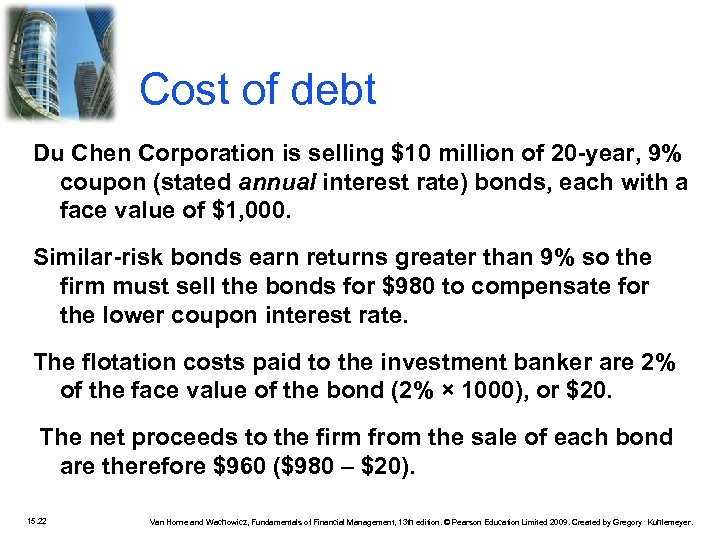Cost of debt Du Chen Corporation is selling \$10 million of 20 -year, 9% coupon (stated annual interest rate) bonds, each with a face value of \$1, 000. Similar-risk bonds earn returns greater than 9% so the firm must sell the bonds for \$980 to compensate for the lower coupon interest rate. The flotation costs paid to the investment banker are 2% of the face value of the bond (2% × 1000), or \$20. The net proceeds to the firm from the sale of each bond are therefore \$960 (\$980 – \$20). 15. 22 Van Horne and Wachowicz, Fundamentals of Financial Management, 13 th edition. © Pearson Education Limited 2009. Created by Gregory Kuhlemeyer.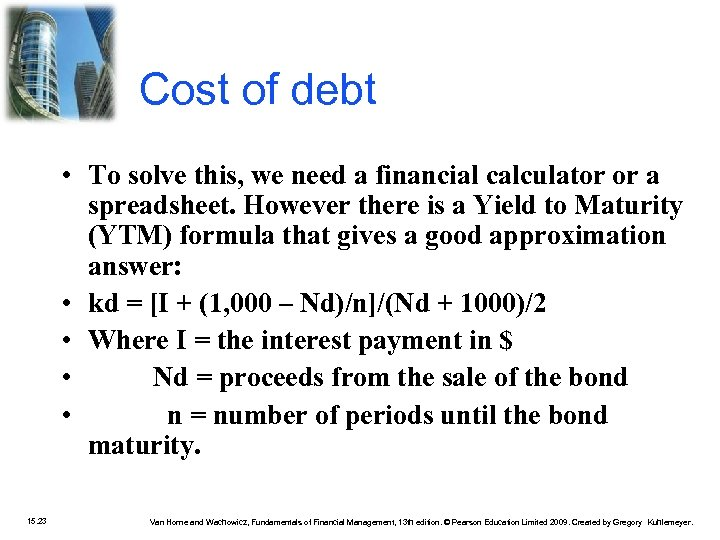Cost of debt • To solve this, we need a financial calculator or a spreadsheet. However there is a Yield to Maturity (YTM) formula that gives a good approximation answer: • kd = [I + (1, 000 – Nd)/n]/(Nd + 1000)/2 • Where I = the interest payment in \$ • Nd = proceeds from the sale of the bond • n = number of periods until the bond maturity. 15. 23 Van Horne and Wachowicz, Fundamentals of Financial Management, 13 th edition. © Pearson Education Limited 2009. Created by Gregory Kuhlemeyer.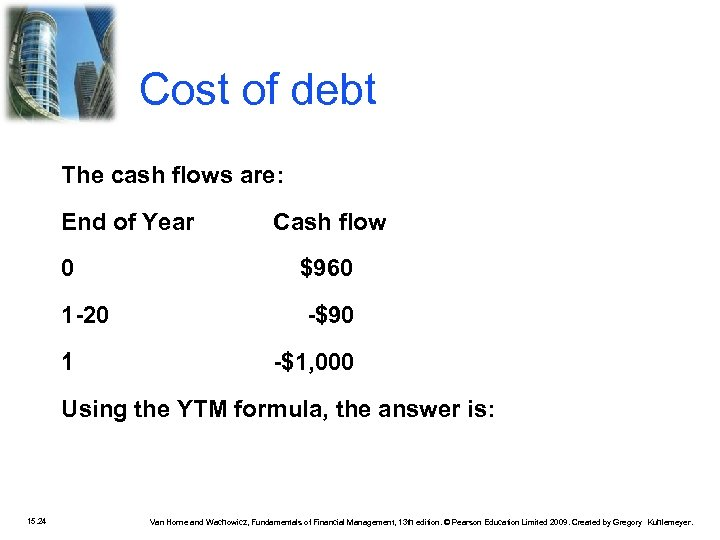Cost of debt The cash flows are: End of Year Cash flow 0 \$960 1 -20 -\$90 1 -\$1, 000 Using the YTM formula, the answer is: 15. 24 Van Horne and Wachowicz, Fundamentals of Financial Management, 13 th edition. © Pearson Education Limited 2009. Created by Gregory Kuhlemeyer.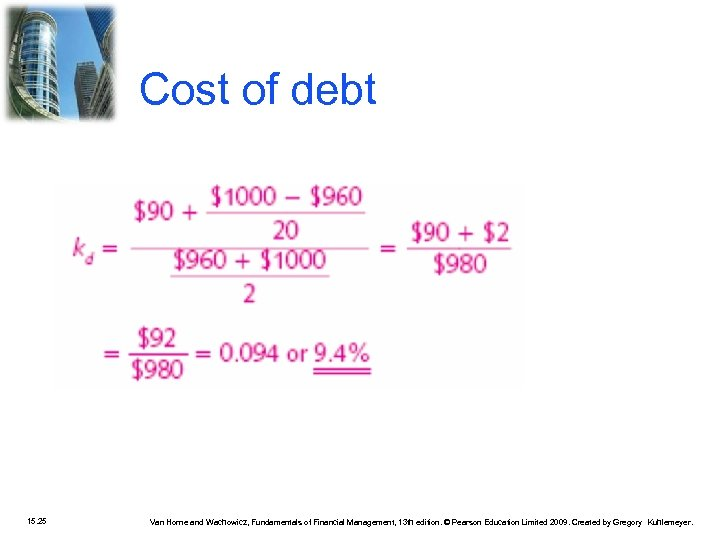Cost of debt 15. 25 Van Horne and Wachowicz, Fundamentals of Financial Management, 13 th edition. © Pearson Education Limited 2009. Created by Gregory Kuhlemeyer.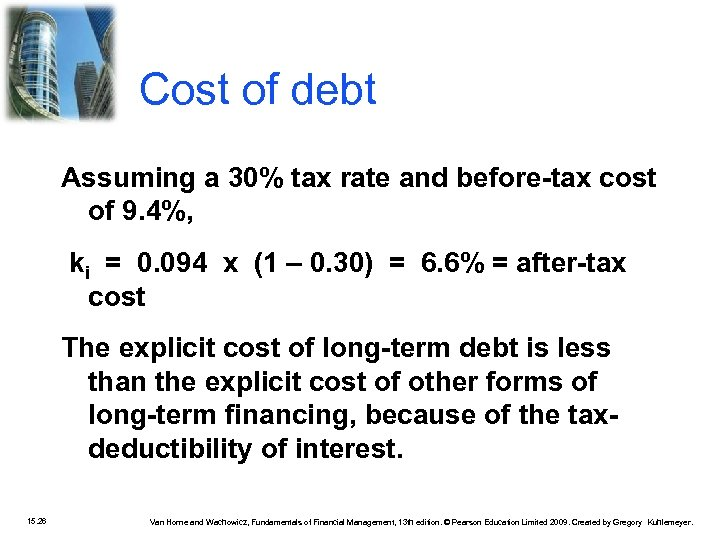Cost of debt Assuming a 30% tax rate and before-tax cost of 9. 4%, ki = 0. 094 x (1 – 0. 30) = 6. 6% = after-tax cost The explicit cost of long-term debt is less than the explicit cost of other forms of long-term financing, because of the taxdeductibility of interest. 15. 26 Van Horne and Wachowicz, Fundamentals of Financial Management, 13 th edition. © Pearson Education Limited 2009. Created by Gregory Kuhlemeyer.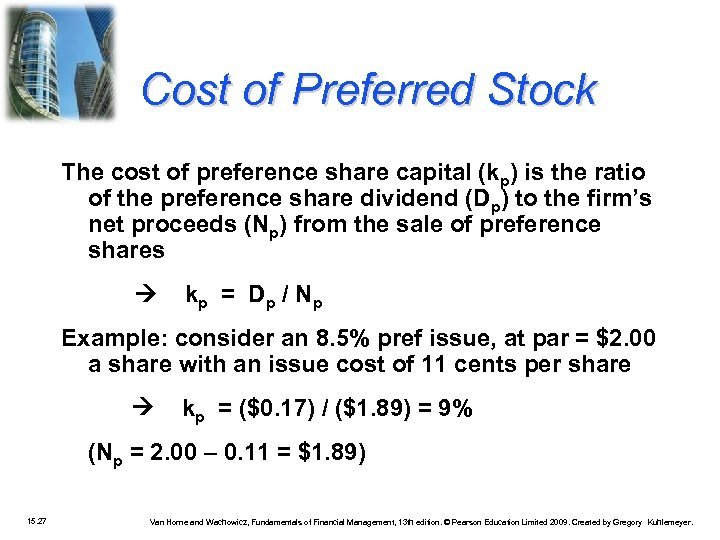Cost of Preferred Stock The cost of preference share capital (kp) is the ratio of the preference share dividend (Dp) to the firm’s net proceeds (Np) from the sale of preference shares k p = D p / Np Example: consider an 8. 5% pref issue, at par = \$2. 00 a share with an issue cost of 11 cents per share kp = (\$0. 17) / (\$1. 89) = 9% (Np = 2. 00 – 0. 11 = \$1. 89) 15. 27 Van Horne and Wachowicz, Fundamentals of Financial Management, 13 th edition. © Pearson Education Limited 2009. Created by Gregory Kuhlemeyer.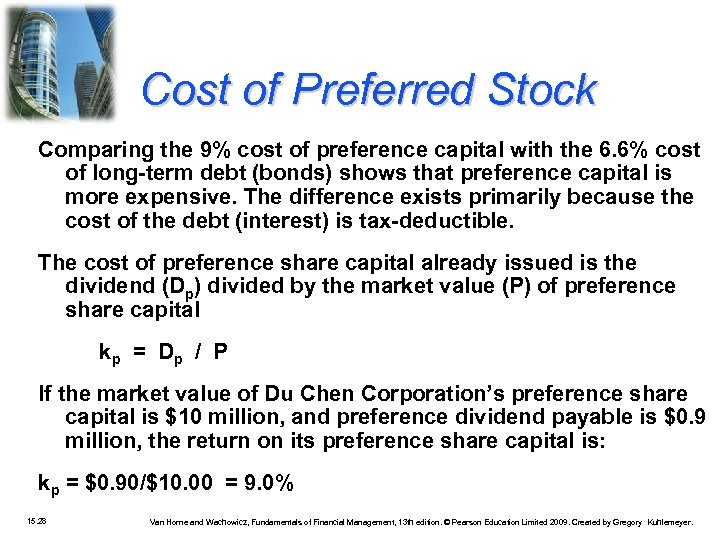Cost of Preferred Stock Comparing the 9% cost of preference capital with the 6. 6% cost of long-term debt (bonds) shows that preference capital is more expensive. The difference exists primarily because the cost of the debt (interest) is tax-deductible. The cost of preference share capital already issued is the dividend (Dp) divided by the market value (P) of preference share capital k p = Dp / P If the market value of Du Chen Corporation’s preference share capital is \$10 million, and preference dividend payable is \$0. 9 million, the return on its preference share capital is: kp = \$0. 90/\$10. 00 = 9. 0% 15. 28 Van Horne and Wachowicz, Fundamentals of Financial Management, 13 th edition. © Pearson Education Limited 2009. Created by Gregory Kuhlemeyer.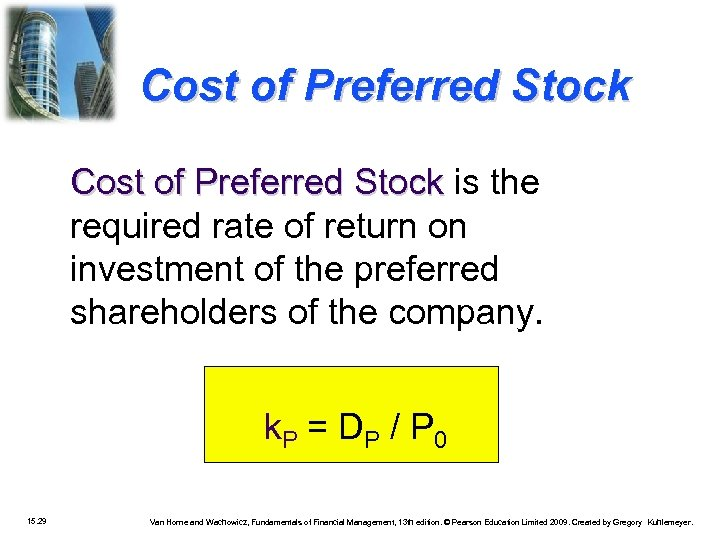Cost of Preferred Stock is the required rate of return on investment of the preferred shareholders of the company. k. P = DP / P 0 15. 29 Van Horne and Wachowicz, Fundamentals of Financial Management, 13 th edition. © Pearson Education Limited 2009. Created by Gregory Kuhlemeyer.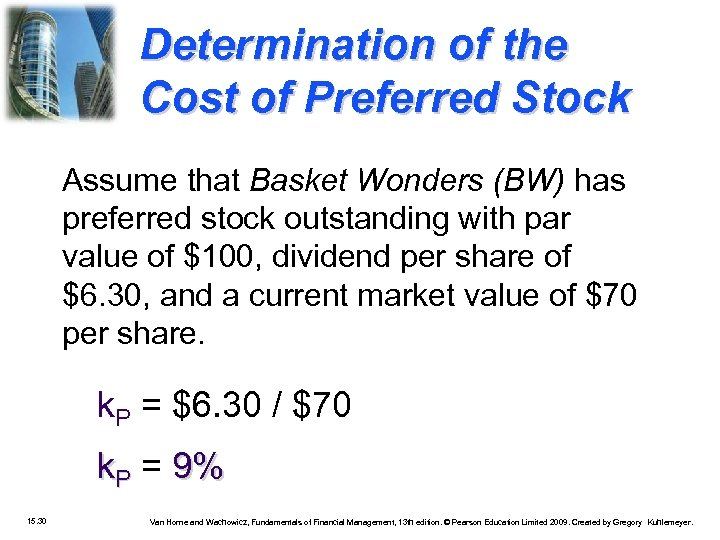Determination of the Cost of Preferred Stock Assume that Basket Wonders (BW) has preferred stock outstanding with par value of \$100, dividend per share of \$6. 30, and a current market value of \$70 per share. k. P = \$6. 30 / \$70 k. P = 9% 15. 30 Van Horne and Wachowicz, Fundamentals of Financial Management, 13 th edition. © Pearson Education Limited 2009. Created by Gregory Kuhlemeyer.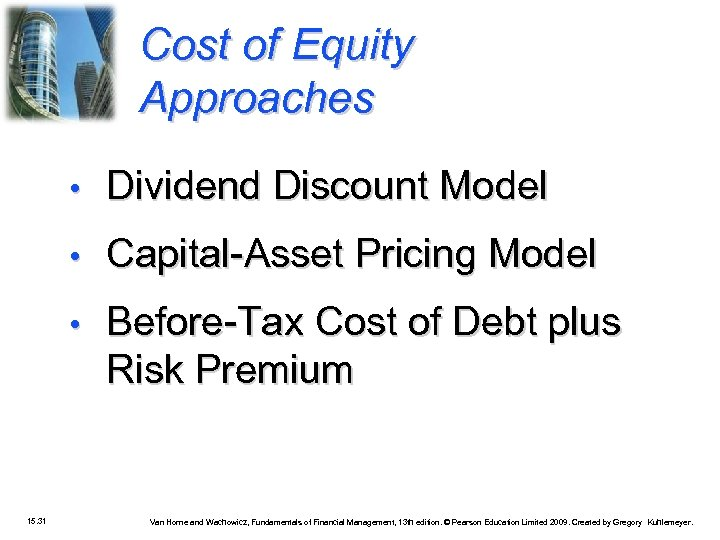Cost of Equity Approaches • • Capital-Asset Pricing Model • 15. 31 Dividend Discount Model Before-Tax Cost of Debt plus Risk Premium Van Horne and Wachowicz, Fundamentals of Financial Management, 13 th edition. © Pearson Education Limited 2009. Created by Gregory Kuhlemeyer.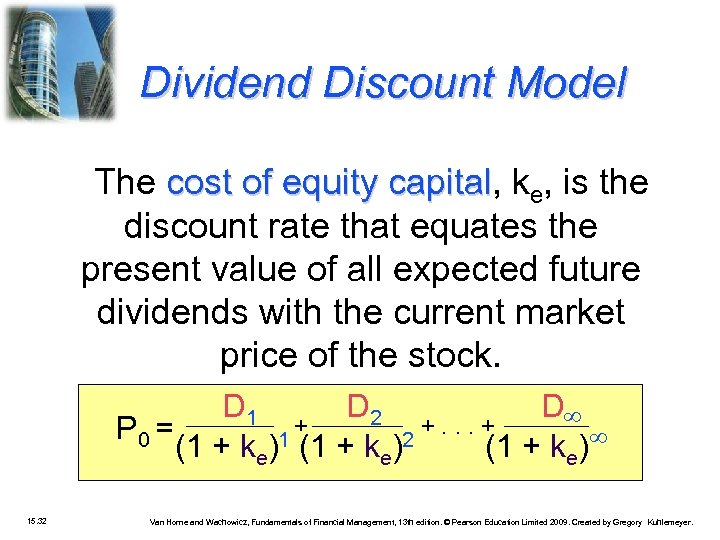Dividend Discount Model The cost of equity capital, ke, is the capital discount rate that equates the present value of all expected future dividends with the current market price of the stock. D 1 D 2 D + +. . . + P 0 = 1 (1 + k )2 (1 + ke) e 15. 32 Van Horne and Wachowicz, Fundamentals of Financial Management, 13 th edition. © Pearson Education Limited 2009. Created by Gregory Kuhlemeyer.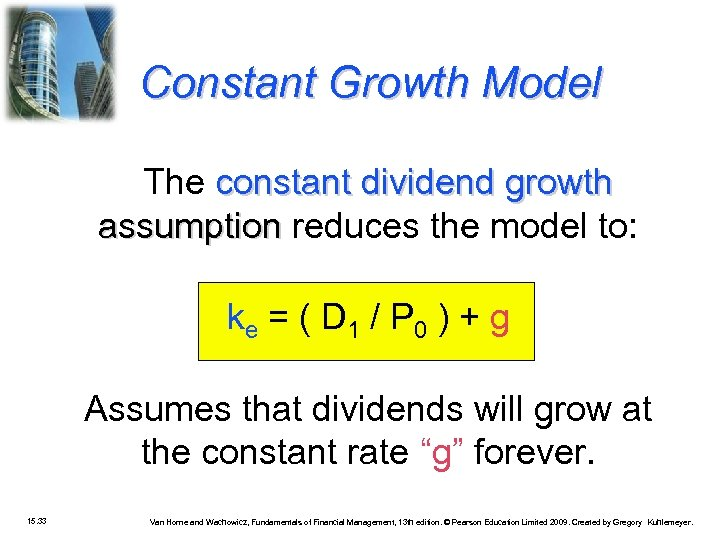Constant Growth Model The constant dividend growth assumption reduces the model to: ke = ( D 1 / P 0 ) + g Assumes that dividends will grow at the constant rate “g” forever. 15. 33 Van Horne and Wachowicz, Fundamentals of Financial Management, 13 th edition. © Pearson Education Limited 2009. Created by Gregory Kuhlemeyer.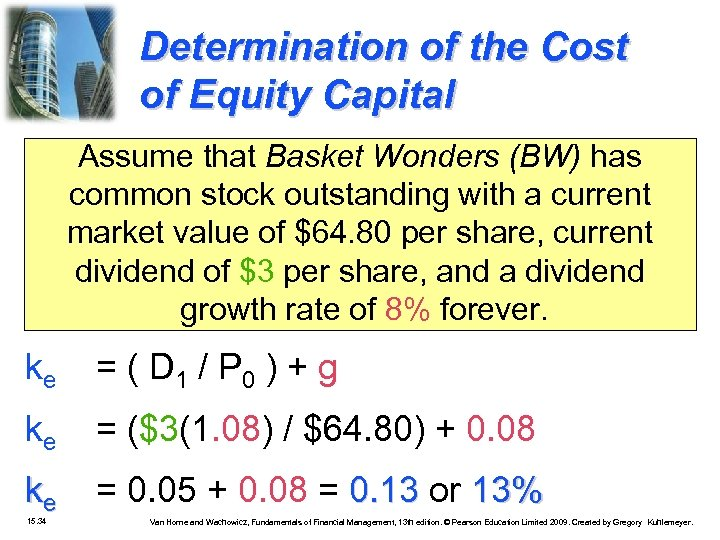Determination of the Cost of Equity Capital Assume that Basket Wonders (BW) has common stock outstanding with a current market value of \$64. 80 per share, current dividend of \$3 per share, and a dividend growth rate of 8% forever. ke = ( D 1 / P 0 ) + g ke = (\$3(1. 08) / \$64. 80) + 0. 08 ke = 0. 05 + 0. 08 = 0. 13 or 13% 15. 34 Van Horne and Wachowicz, Fundamentals of Financial Management, 13 th edition. © Pearson Education Limited 2009. Created by Gregory Kuhlemeyer.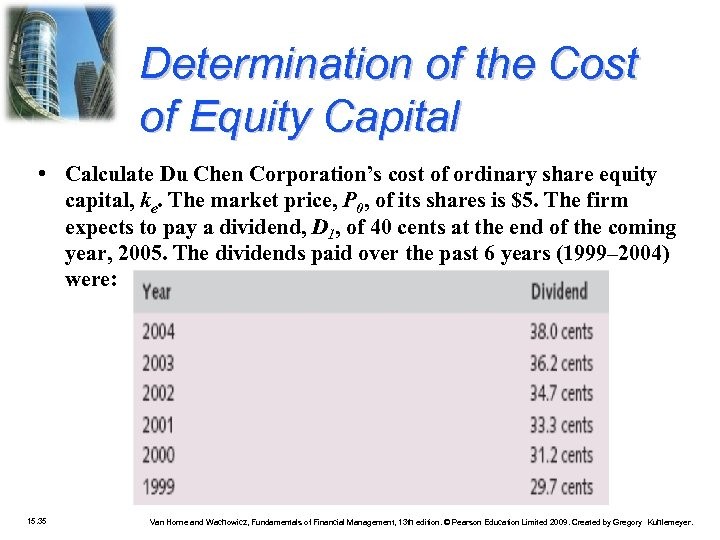Determination of the Cost of Equity Capital • Calculate Du Chen Corporation’s cost of ordinary share equity capital, ke. The market price, P 0, of its shares is \$5. The firm expects to pay a dividend, D 1, of 40 cents at the end of the coming year, 2005. The dividends paid over the past 6 years (1999– 2004) were: 15. 35 Van Horne and Wachowicz, Fundamentals of Financial Management, 13 th edition. © Pearson Education Limited 2009. Created by Gregory Kuhlemeyer.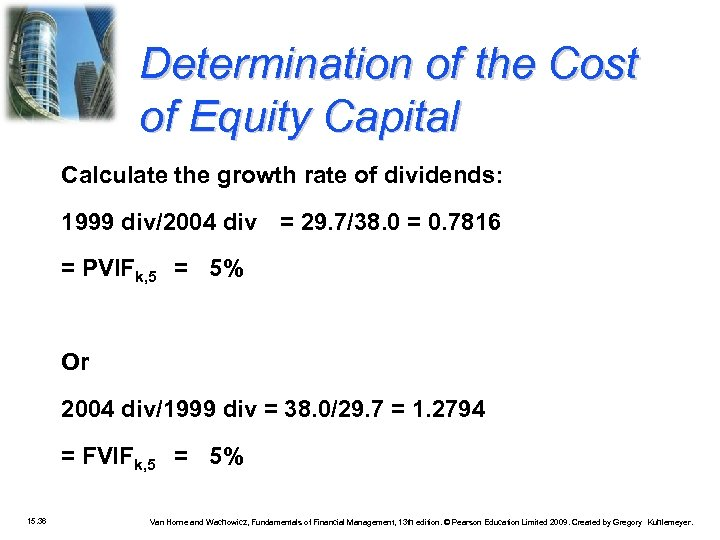Determination of the Cost of Equity Capital Calculate the growth rate of dividends: 1999 div/2004 div = 29. 7/38. 0 = 0. 7816 = PVIFk, 5 = 5% Or 2004 div/1999 div = 38. 0/29. 7 = 1. 2794 = FVIFk, 5 = 5% 15. 36 Van Horne and Wachowicz, Fundamentals of Financial Management, 13 th edition. © Pearson Education Limited 2009. Created by Gregory Kuhlemeyer.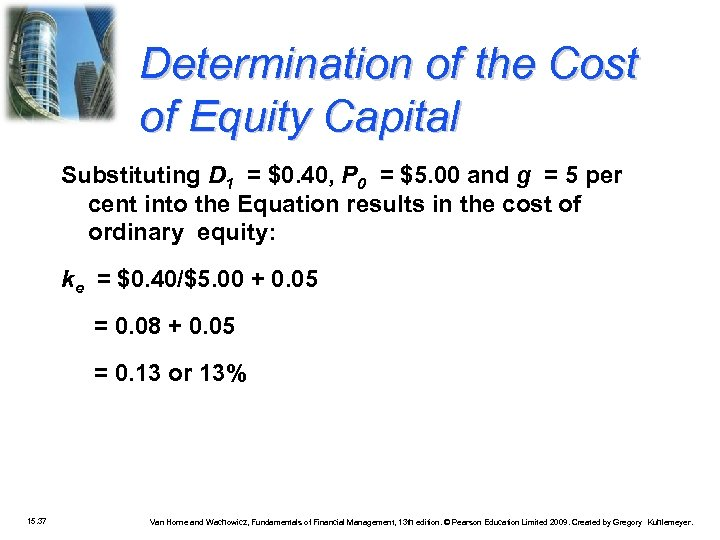Determination of the Cost of Equity Capital Substituting D 1 = \$0. 40, P 0 = \$5. 00 and g = 5 per cent into the Equation results in the cost of ordinary equity: ke = \$0. 40/\$5. 00 + 0. 05 = 0. 08 + 0. 05 = 0. 13 or 13% 15. 37 Van Horne and Wachowicz, Fundamentals of Financial Management, 13 th edition. © Pearson Education Limited 2009. Created by Gregory Kuhlemeyer.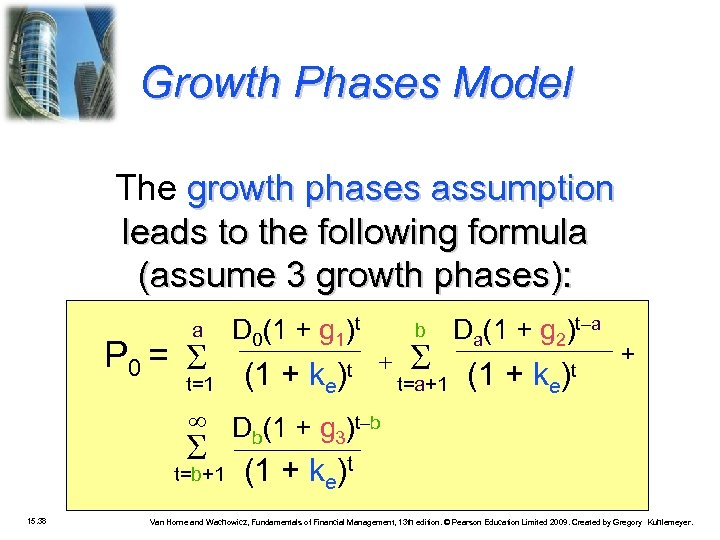Growth Phases Model The growth phases assumption leads to the following formula (assume 3 growth phases): a D 0(1 + g 1)t t=1 (1 + ke)t P 0 = t=b+1 15. 38 b Da(1 + g 2)t–a t=a+1 (1 + ke)t + Db(1 + g 3)t–b (1 + ke)t Van Horne and Wachowicz, Fundamentals of Financial Management, 13 th edition. © Pearson Education Limited 2009. Created by Gregory Kuhlemeyer.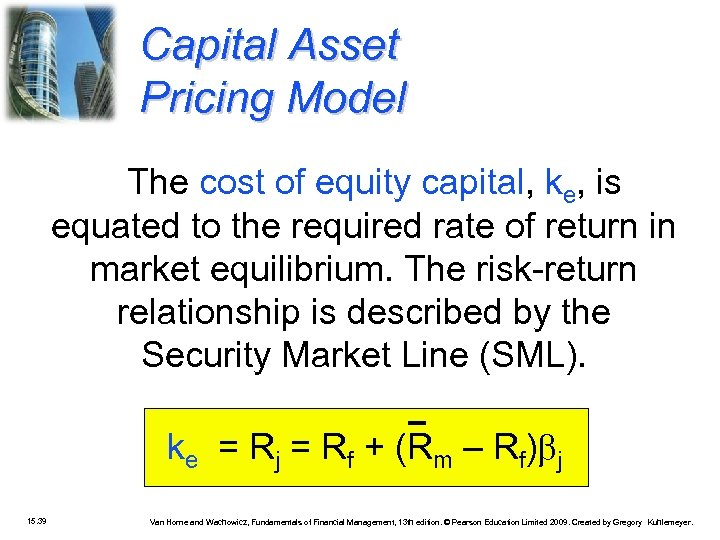Capital Asset Pricing Model The cost of equity capital, ke, is equated to the required rate of return in market equilibrium. The risk-return relationship is described by the Security Market Line (SML). ke = Rj = Rf + (Rm – Rf) j 15. 39 Van Horne and Wachowicz, Fundamentals of Financial Management, 13 th edition. © Pearson Education Limited 2009. Created by Gregory Kuhlemeyer.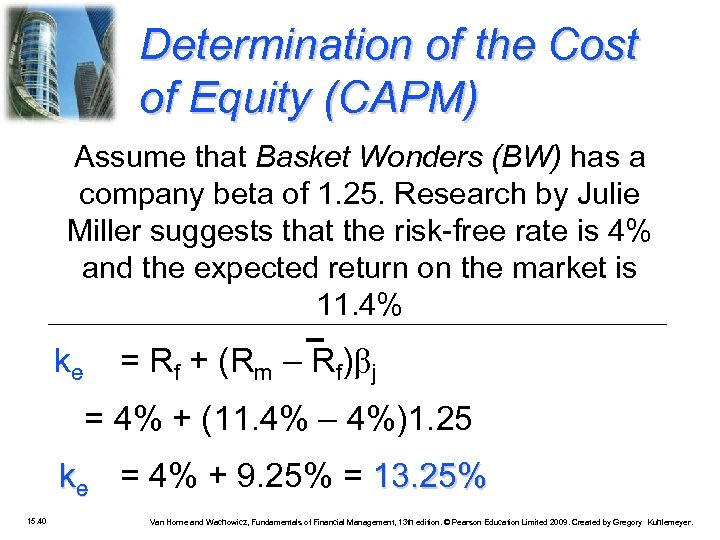Determination of the Cost of Equity (CAPM) Assume that Basket Wonders (BW) has a company beta of 1. 25. Research by Julie Miller suggests that the risk-free rate is 4% and the expected return on the market is 11. 4% ke = Rf + (Rm – Rf) j = 4% + (11. 4% – 4%)1. 25 ke = 4% + 9. 25% = 13. 25% 15. 40 Van Horne and Wachowicz, Fundamentals of Financial Management, 13 th edition. © Pearson Education Limited 2009. Created by Gregory Kuhlemeyer.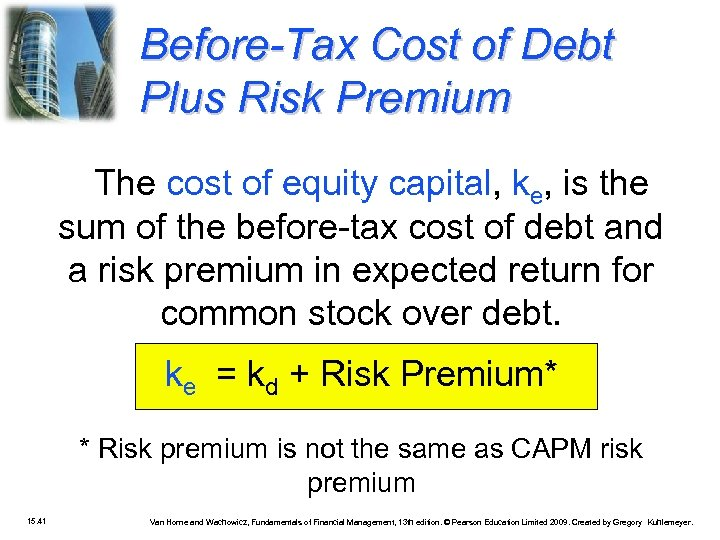Before-Tax Cost of Debt Plus Risk Premium The cost of equity capital, ke, is the sum of the before-tax cost of debt and a risk premium in expected return for common stock over debt. ke = kd + Risk Premium* * Risk premium is not the same as CAPM risk premium 15. 41 Van Horne and Wachowicz, Fundamentals of Financial Management, 13 th edition. © Pearson Education Limited 2009. Created by Gregory Kuhlemeyer.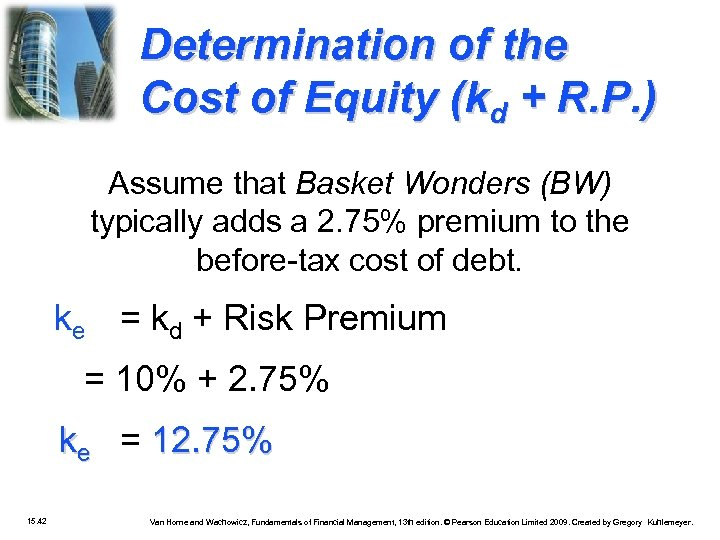Determination of the Cost of Equity (kd + R. P. ) Assume that Basket Wonders (BW) typically adds a 2. 75% premium to the before-tax cost of debt. ke = kd + Risk Premium = 10% + 2. 75% ke = 12. 75% 15. 42 Van Horne and Wachowicz, Fundamentals of Financial Management, 13 th edition. © Pearson Education Limited 2009. Created by Gregory Kuhlemeyer.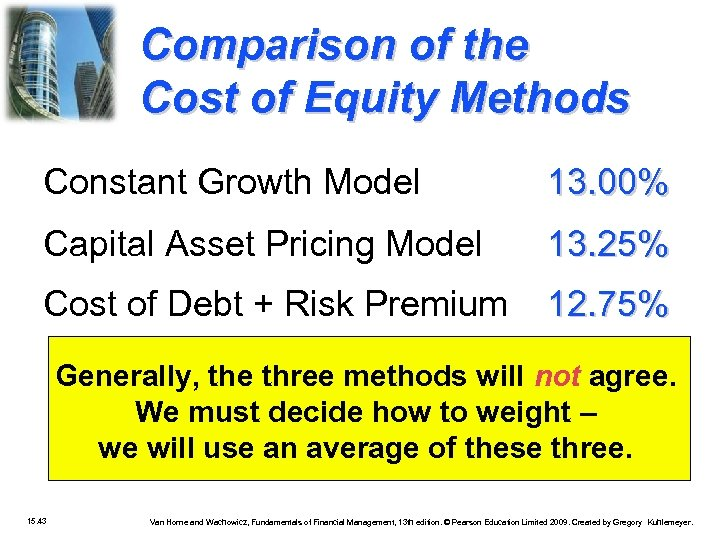Comparison of the Cost of Equity Methods Constant Growth Model 13. 00% Capital Asset Pricing Model 13. 25% Cost of Debt + Risk Premium 12. 75% Generally, the three methods will not agree. We must decide how to weight – we will use an average of these three. 15. 43 Van Horne and Wachowicz, Fundamentals of Financial Management, 13 th edition. © Pearson Education Limited 2009. Created by Gregory Kuhlemeyer.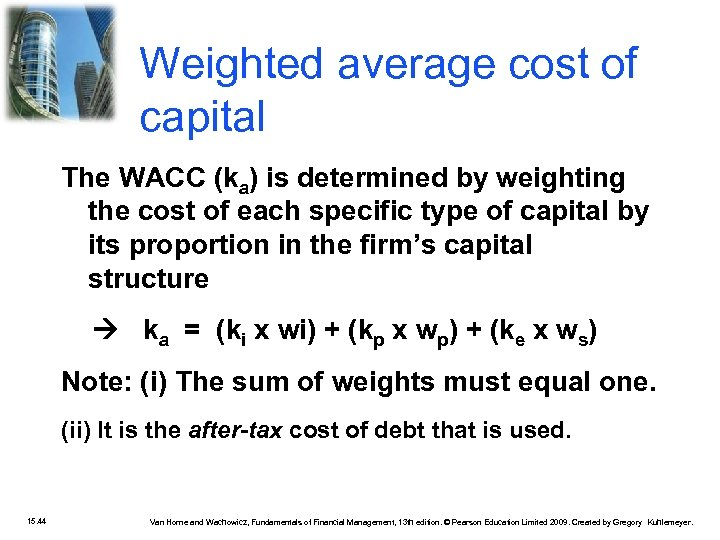Weighted average cost of capital The WACC (ka) is determined by weighting the cost of each specific type of capital by its proportion in the firm’s capital structure ka = (ki x wi) + (kp x wp) + (ke x ws) Note: (i) The sum of weights must equal one. (ii) It is the after-tax cost of debt that is used. 15. 44 Van Horne and Wachowicz, Fundamentals of Financial Management, 13 th edition. © Pearson Education Limited 2009. Created by Gregory Kuhlemeyer.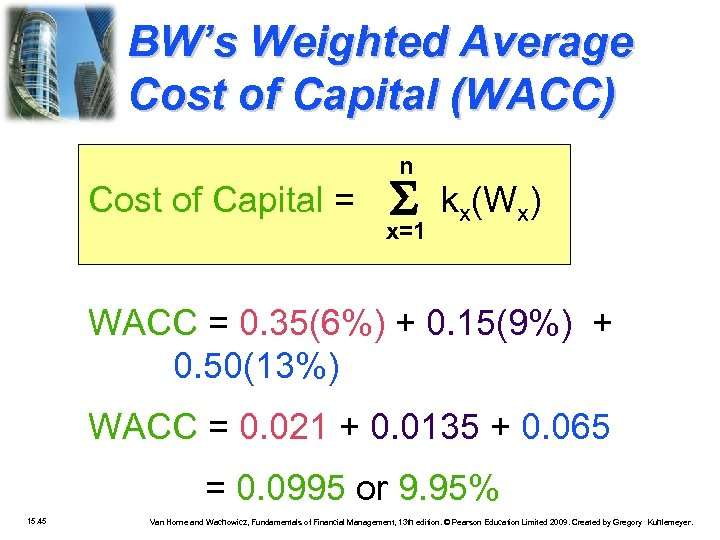BW’s Weighted Average Cost of Capital (WACC) n Cost of Capital = x=1 kx(Wx) WACC = 0. 35(6%) + 0. 15(9%) + 0. 50(13%) WACC = 0. 021 + 0. 0135 + 0. 065 = 0. 0995 or 9. 95% 15. 45 Van Horne and Wachowicz, Fundamentals of Financial Management, 13 th edition. © Pearson Education Limited 2009. Created by Gregory Kuhlemeyer.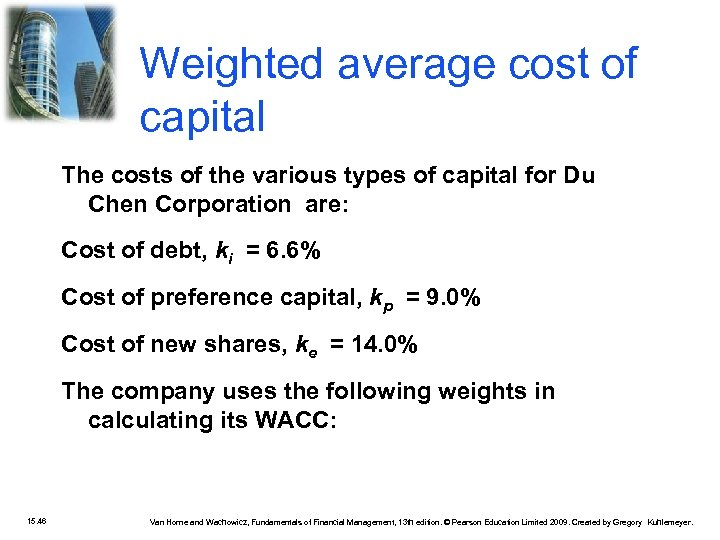Weighted average cost of capital The costs of the various types of capital for Du Chen Corporation are: Cost of debt, ki = 6. 6% Cost of preference capital, kp = 9. 0% Cost of new shares, ke = 14. 0% The company uses the following weights in calculating its WACC: 15. 46 Van Horne and Wachowicz, Fundamentals of Financial Management, 13 th edition. © Pearson Education Limited 2009. Created by Gregory Kuhlemeyer.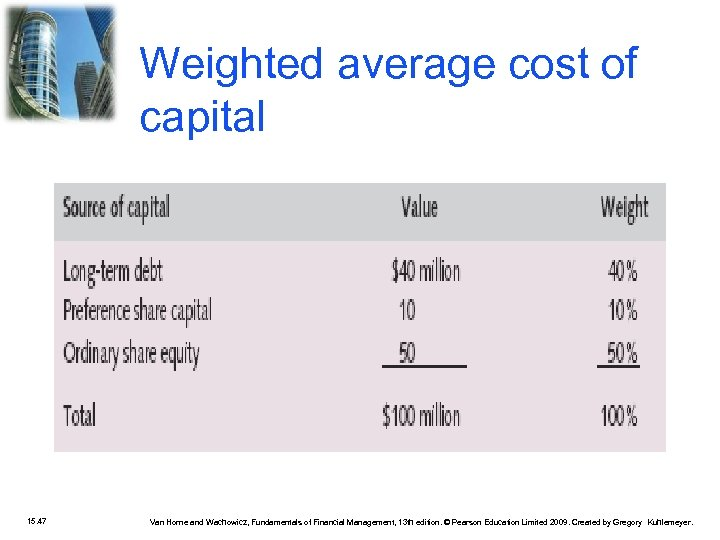Weighted average cost of capital 15. 47 Van Horne and Wachowicz, Fundamentals of Financial Management, 13 th edition. © Pearson Education Limited 2009. Created by Gregory Kuhlemeyer.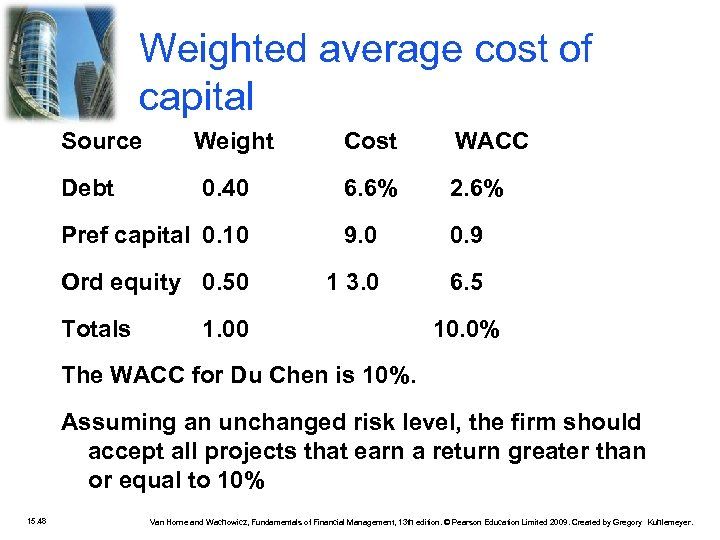Weighted average cost of capital Source Cost WACC 6. 6% 2. 6% Pref capital 0. 10 9. 0 0. 9 Ord equity 0. 50 1 3. 0 6. 5 Debt Totals Weight 0. 40 1. 00 10. 0% The WACC for Du Chen is 10%. Assuming an unchanged risk level, the firm should accept all projects that earn a return greater than or equal to 10% 15. 48 Van Horne and Wachowicz, Fundamentals of Financial Management, 13 th edition. © Pearson Education Limited 2009. Created by Gregory Kuhlemeyer.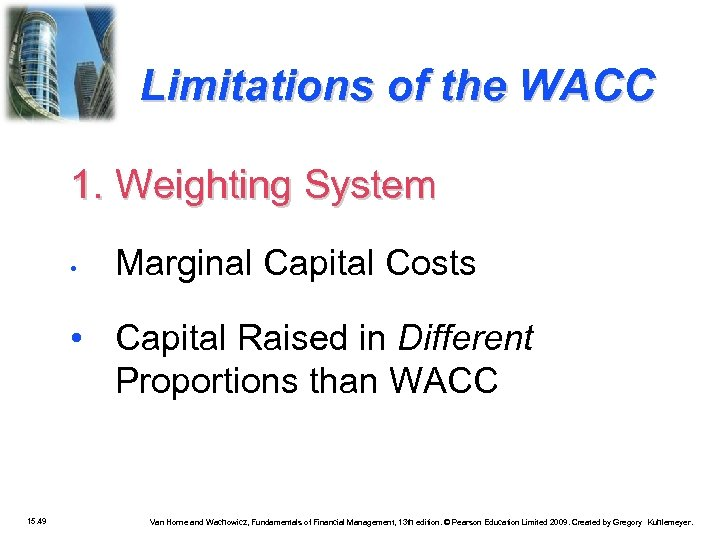Limitations of the WACC 1. Weighting System • Marginal Capital Costs • Capital Raised in Different Proportions than WACC 15. 49 Van Horne and Wachowicz, Fundamentals of Financial Management, 13 th edition. © Pearson Education Limited 2009. Created by Gregory Kuhlemeyer.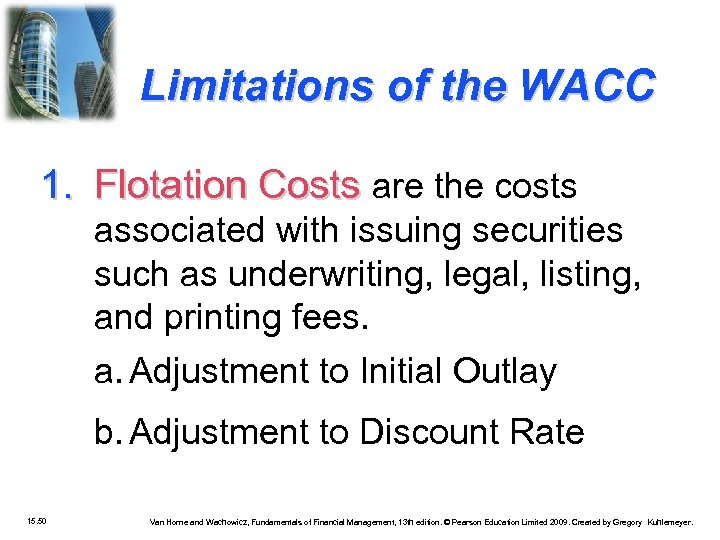Limitations of the WACC 1. Flotation Costs are the costs associated with issuing securities such as underwriting, legal, listing, and printing fees. a. Adjustment to Initial Outlay b. Adjustment to Discount Rate 15. 50 Van Horne and Wachowicz, Fundamentals of Financial Management, 13 th edition. © Pearson Education Limited 2009. Created by Gregory Kuhlemeyer.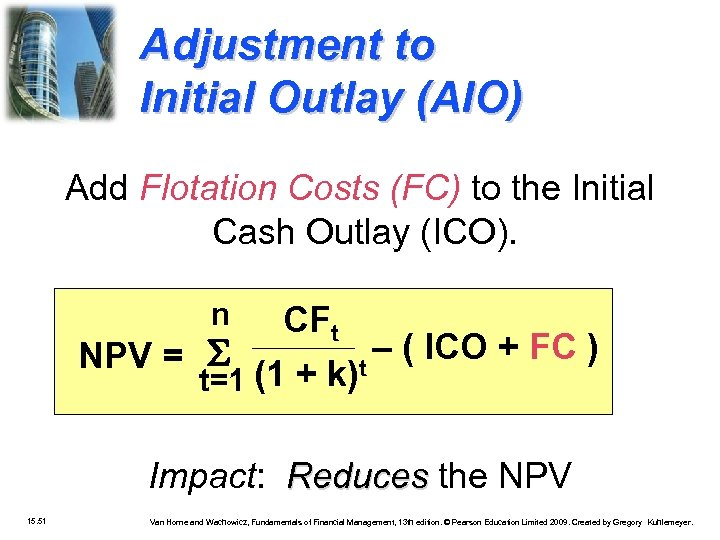Adjustment to Initial Outlay (AIO) Add Flotation Costs (FC) to the Initial Cash Outlay (ICO). n CFt NPV = (1 + k)t – ( ICO + FC ) t=1 Impact: Reduces the NPV 15. 51 Van Horne and Wachowicz, Fundamentals of Financial Management, 13 th edition. © Pearson Education Limited 2009. Created by Gregory Kuhlemeyer.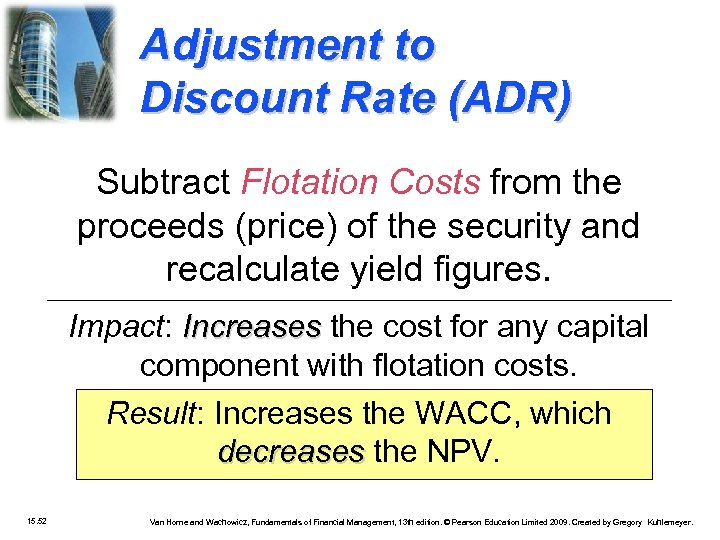Adjustment to Discount Rate (ADR) Subtract Flotation Costs from the proceeds (price) of the security and recalculate yield figures. Impact: Increases the cost for any capital component with flotation costs. Result: Increases the WACC, which decreases the NPV. 15. 52 Van Horne and Wachowicz, Fundamentals of Financial Management, 13 th edition. © Pearson Education Limited 2009. Created by Gregory Kuhlemeyer.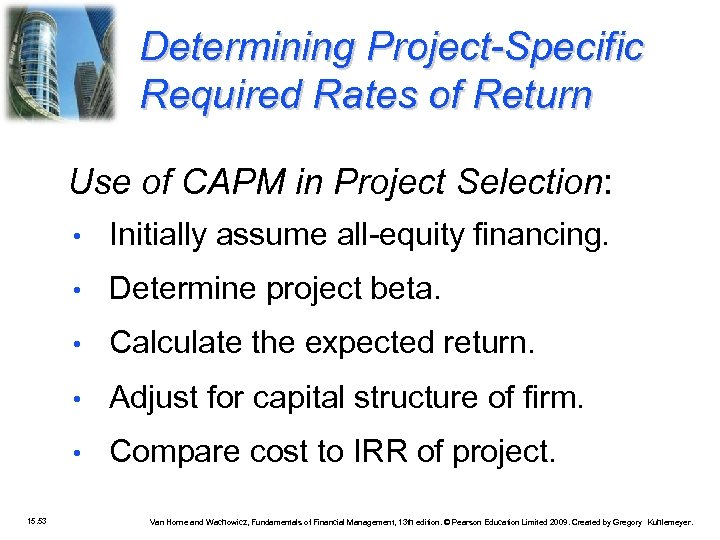Determining Project-Specific Required Rates of Return Use of CAPM in Project Selection: • • Determine project beta. • Calculate the expected return. • Adjust for capital structure of firm. • 15. 53 Initially assume all-equity financing. Compare cost to IRR of project. Van Horne and Wachowicz, Fundamentals of Financial Management, 13 th edition. © Pearson Education Limited 2009. Created by Gregory Kuhlemeyer.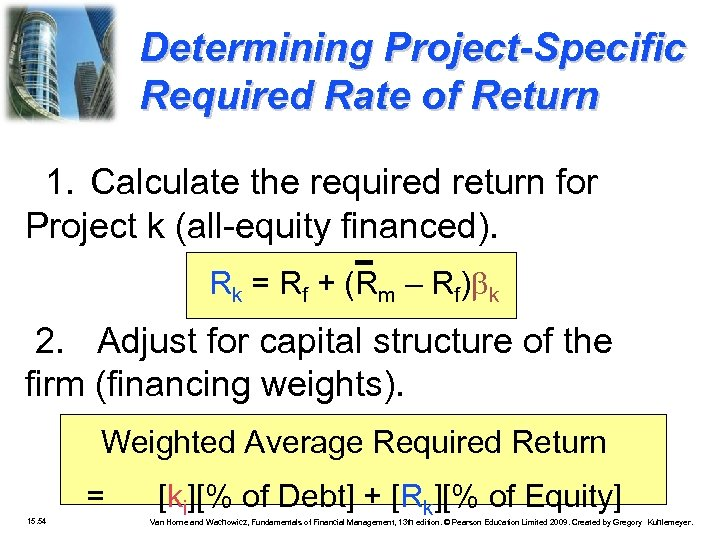Determining Project-Specific Required Rate of Return 1. Calculate the required return for Project k (all-equity financed). Rk = Rf + (Rm – Rf) k 2. Adjust for capital structure of the firm (financing weights). Weighted Average Required Return = 15. 54 [ki][% of Debt] + [Rk][% of Equity] Van Horne and Wachowicz, Fundamentals of Financial Management, 13 th edition. © Pearson Education Limited 2009. Created by Gregory Kuhlemeyer.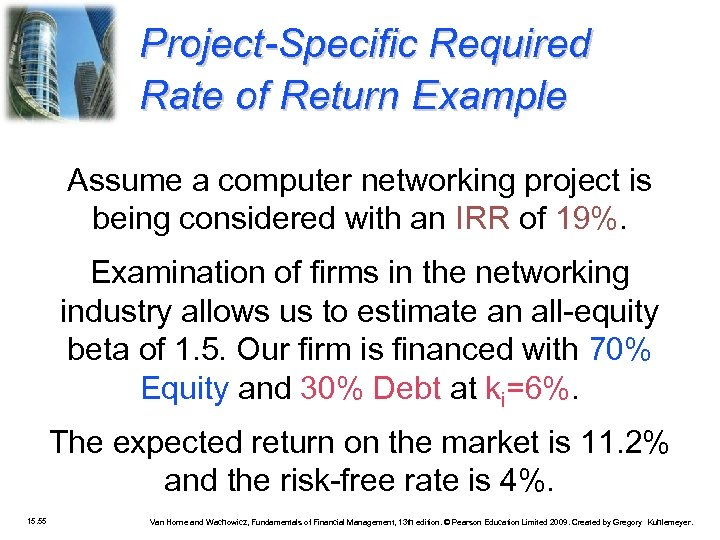Project-Specific Required Rate of Return Example Assume a computer networking project is being considered with an IRR of 19%. Examination of firms in the networking industry allows us to estimate an all-equity beta of 1. 5. Our firm is financed with 70% Equity and 30% Debt at ki=6%. The expected return on the market is 11. 2% and the risk-free rate is 4%. 15. 55 Van Horne and Wachowicz, Fundamentals of Financial Management, 13 th edition. © Pearson Education Limited 2009. Created by Gregory Kuhlemeyer.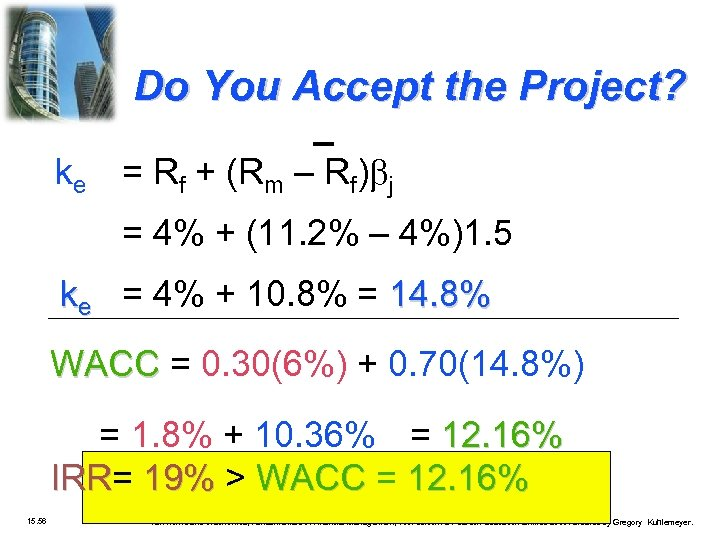Do You Accept the Project? ke = Rf + (Rm – Rf) j = 4% + (11. 2% – 4%)1. 5 ke = 4% + 10. 8% = 14. 8% WACC = 0. 30(6%) + 0. 70(14. 8%) = 1. 8% + 10. 36% = 12. 16% IRR= 19% > WACC = 12. 16% IRR 15. 56 Van Horne and Wachowicz, Fundamentals of Financial Management, 13 th edition. © Pearson Education Limited 2009. Created by Gregory Kuhlemeyer.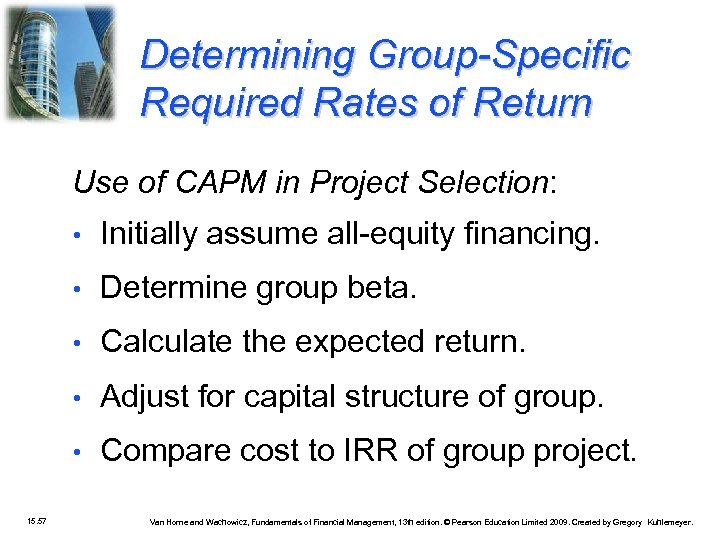Determining Group-Specific Required Rates of Return Use of CAPM in Project Selection: • • Determine group beta. • Calculate the expected return. • Adjust for capital structure of group. • 15. 57 Initially assume all-equity financing. Compare cost to IRR of group project. Van Horne and Wachowicz, Fundamentals of Financial Management, 13 th edition. © Pearson Education Limited 2009. Created by Gregory Kuhlemeyer.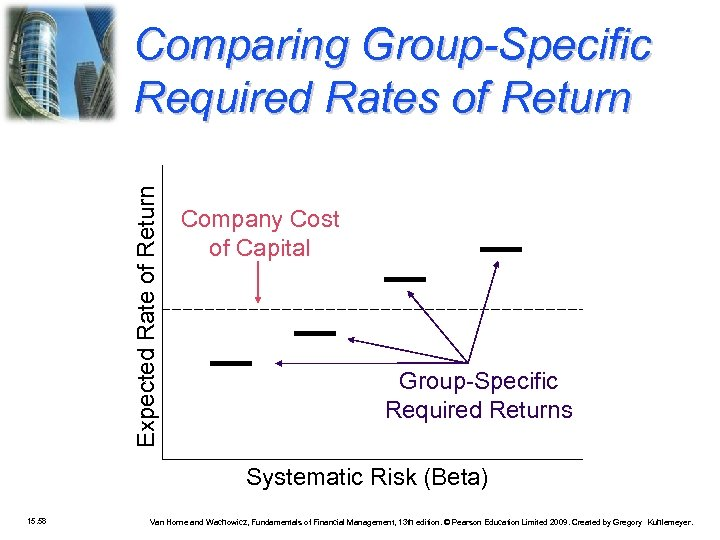Expected Rate of Return Comparing Group-Specific Required Rates of Return Company Cost of Capital Group-Specific Required Returns Systematic Risk (Beta) 15. 58 Van Horne and Wachowicz, Fundamentals of Financial Management, 13 th edition. © Pearson Education Limited 2009. Created by Gregory Kuhlemeyer.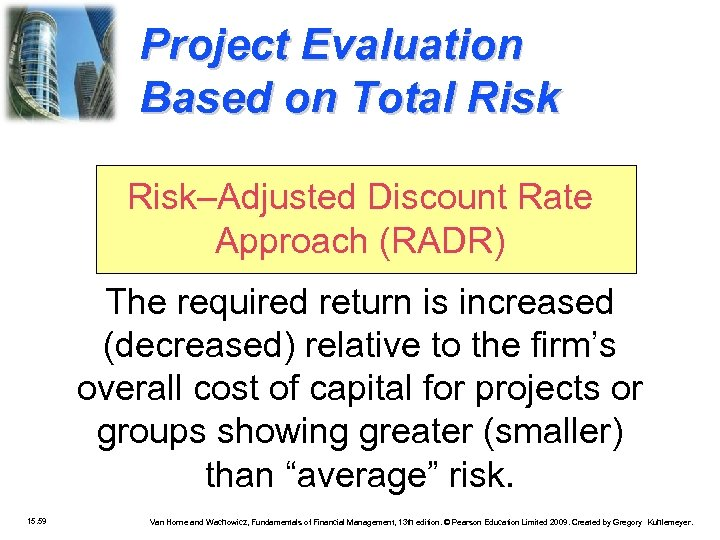Project Evaluation Based on Total Risk–Adjusted Discount Rate Approach (RADR) The required return is increased (decreased) relative to the firm’s overall cost of capital for projects or groups showing greater (smaller) than “average” risk. 15. 59 Van Horne and Wachowicz, Fundamentals of Financial Management, 13 th edition. © Pearson Education Limited 2009. Created by Gregory Kuhlemeyer.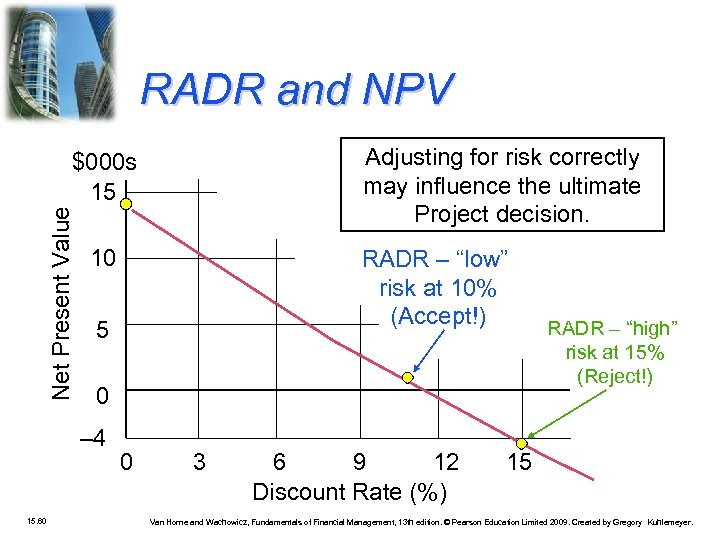RADR and NPV Adjusting for risk correctly may influence the ultimate Project decision. Net Present Value \$000 s 15 10 5 0 – 4 15. 60 RADR – “low” risk at 10% (Accept!) 0 3 6 9 12 Discount Rate (%) RADR – “high” risk at 15% (Reject!) 15 Van Horne and Wachowicz, Fundamentals of Financial Management, 13 th edition. © Pearson Education Limited 2009. Created by Gregory Kuhlemeyer.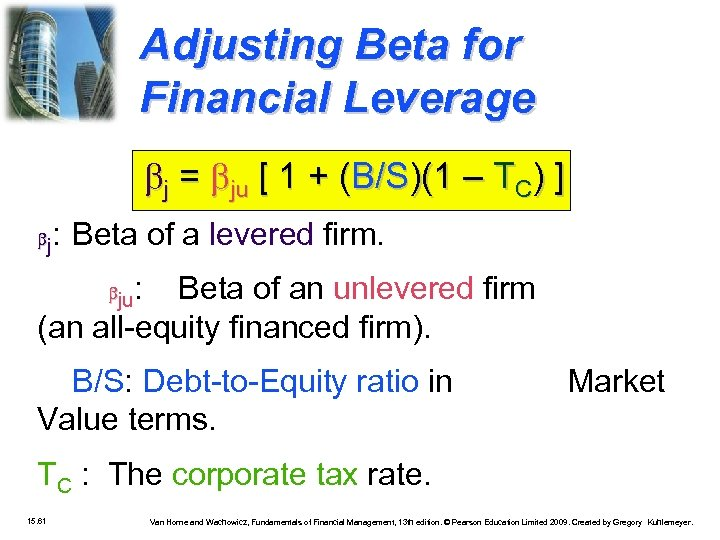Adjusting Beta for Financial Leverage j = ju [ 1 + (B/S)(1 – TC) ] j : Beta of a levered firm. : Beta of an unlevered firm (an all-equity financed firm). ju B/S: Debt-to-Equity ratio in Value terms. Market TC : The corporate tax rate. 15. 61 Van Horne and Wachowicz, Fundamentals of Financial Management, 13 th edition. © Pearson Education Limited 2009. Created by Gregory Kuhlemeyer.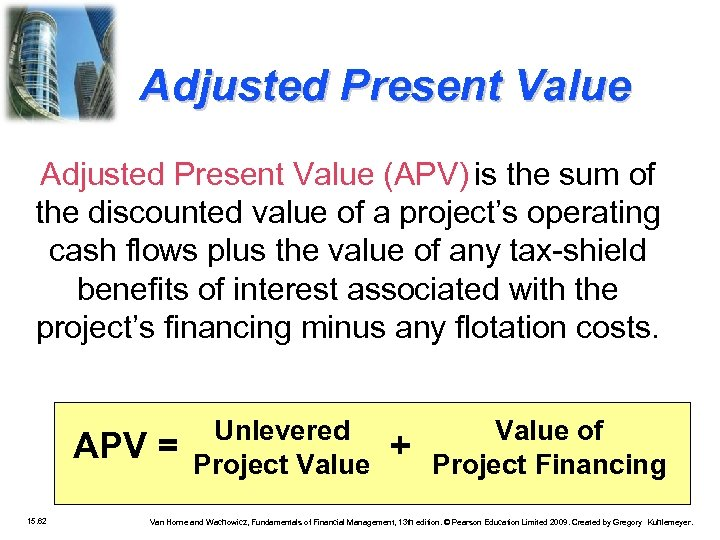Adjusted Present Value (APV) is the sum of the discounted value of a project’s operating cash flows plus the value of any tax-shield benefits of interest associated with the project’s financing minus any flotation costs. APV = 15. 62 Unlevered Project Value + Value of Project Financing Van Horne and Wachowicz, Fundamentals of Financial Management, 13 th edition. © Pearson Education Limited 2009. Created by Gregory Kuhlemeyer.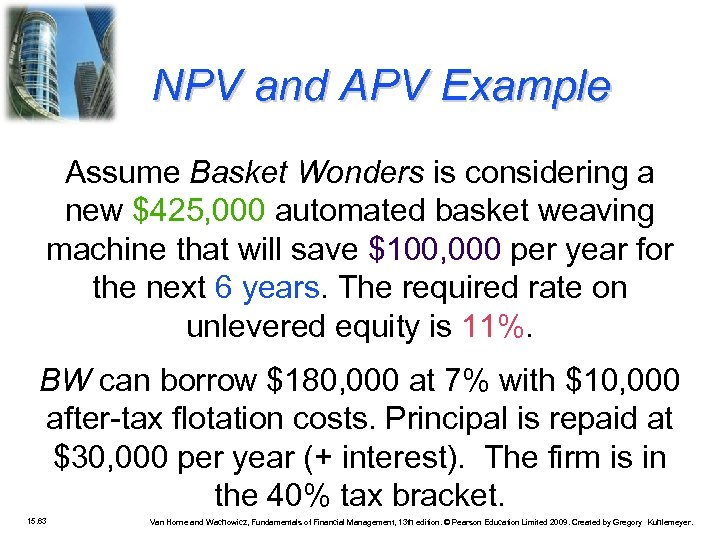NPV and APV Example Assume Basket Wonders is considering a new \$425, 000 automated basket weaving machine that will save \$100, 000 per year for the next 6 years. The required rate on unlevered equity is 11%. BW can borrow \$180, 000 at 7% with \$10, 000 after-tax flotation costs. Principal is repaid at \$30, 000 per year (+ interest). The firm is in the 40% tax bracket. 15. 63 Van Horne and Wachowicz, Fundamentals of Financial Management, 13 th edition. © Pearson Education Limited 2009. Created by Gregory Kuhlemeyer.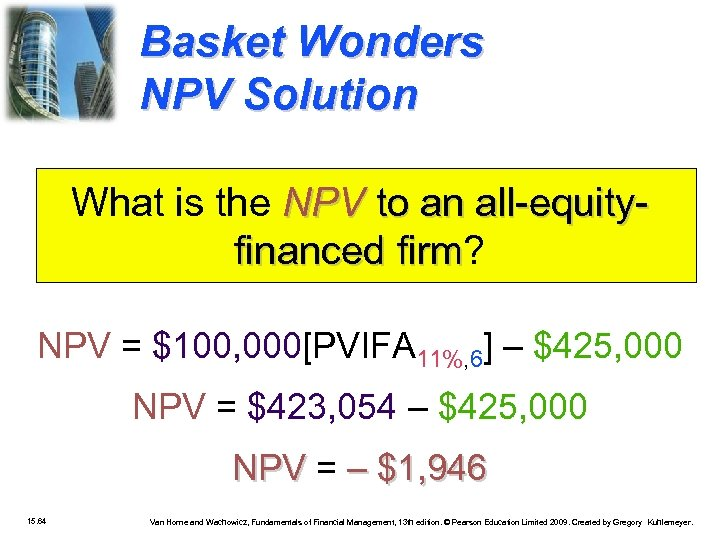Basket Wonders NPV Solution What is the NPV to an all-equityfinanced firm? firm NPV = \$100, 000[PVIFA 11%, 6] – \$425, 000 NPV = \$423, 054 – \$425, 000 NPV = – \$1, 946 15. 64 Van Horne and Wachowicz, Fundamentals of Financial Management, 13 th edition. © Pearson Education Limited 2009. Created by Gregory Kuhlemeyer.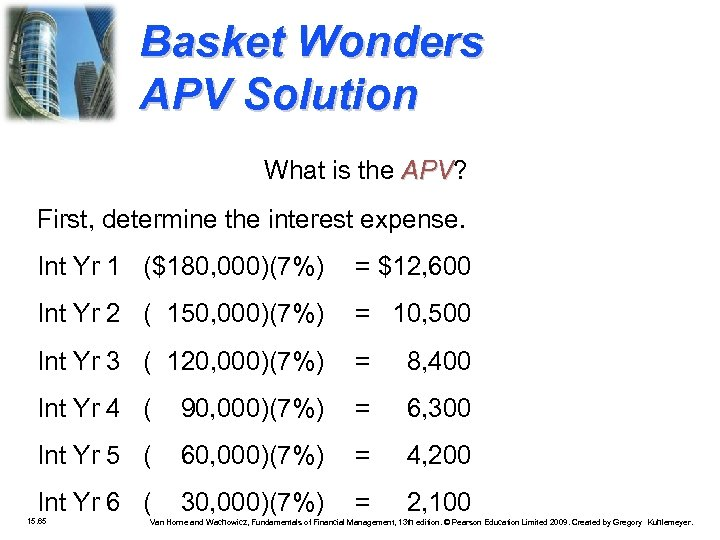Basket Wonders APV Solution What is the APV? APV First, determine the interest expense. Int Yr 1 (\$180, 000)(7%) = \$12, 600 Int Yr 2 ( 150, 000)(7%) = 10, 500 Int Yr 3 ( 120, 000)(7%) = 8, 400 Int Yr 4 ( 90, 000)(7%) = 6, 300 Int Yr 5 ( 60, 000)(7%) = 4, 200 Int Yr 6 ( 30, 000)(7%) = 2, 100 15. 65 Van Horne and Wachowicz, Fundamentals of Financial Management, 13 th edition. © Pearson Education Limited 2009. Created by Gregory Kuhlemeyer.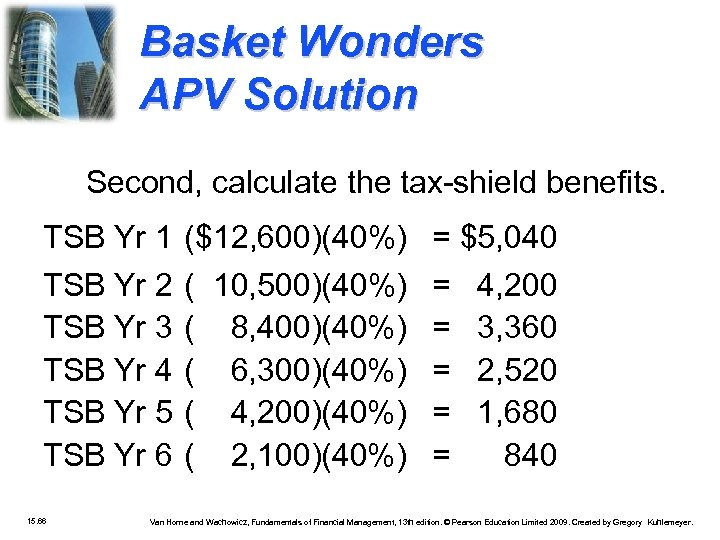Basket Wonders APV Solution Second, calculate the tax-shield benefits. TSB Yr 1 (\$12, 600)(40%) = \$5, 040 TSB Yr 2 TSB Yr 3 TSB Yr 4 TSB Yr 5 TSB Yr 6 15. 66 ( 10, 500)(40%) = 4, 200 ( 8, 400)(40%) = 3, 360 ( 6, 300)(40%) = 2, 520 ( 4, 200)(40%) = 1, 680 ( 2, 100)(40%) = 840 Van Horne and Wachowicz, Fundamentals of Financial Management, 13 th edition. © Pearson Education Limited 2009. Created by Gregory Kuhlemeyer.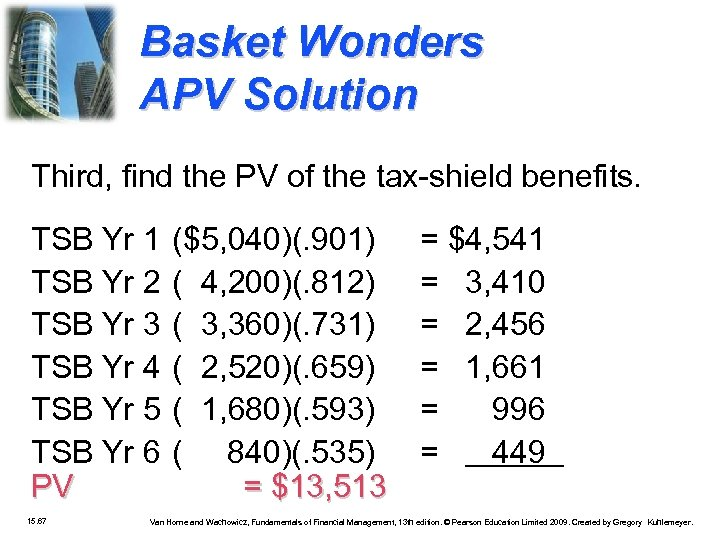Basket Wonders APV Solution Third, find the PV of the tax-shield benefits. TSB Yr 1 TSB Yr 2 TSB Yr 3 TSB Yr 4 TSB Yr 5 TSB Yr 6 PV 15. 67 (\$5, 040)(. 901) ( 4, 200)(. 812) ( 3, 360)(. 731) ( 2, 520)(. 659) ( 1, 680)(. 593) ( 840)(. 535) = \$13, 513 = \$4, 541 = 3, 410 = 2, 456 = 1, 661 = 996 = 449 Van Horne and Wachowicz, Fundamentals of Financial Management, 13 th edition. © Pearson Education Limited 2009. Created by Gregory Kuhlemeyer.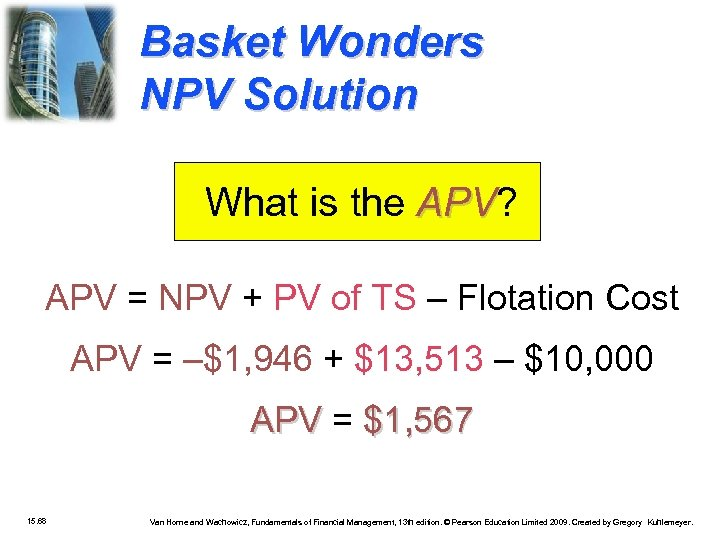Basket Wonders NPV Solution What is the APV? APV = NPV + PV of TS – Flotation Cost APV = –\$1, 946 + \$13, 513 – \$10, 000 APV = \$1, 567 15. 68 Van Horne and Wachowicz, Fundamentals of Financial Management, 13 th edition. © Pearson Education Limited 2009. Created by Gregory Kuhlemeyer.